• 解析几何 版本：2020-03-23 此版本是最终版本。 如有错误请指出，转载时请注明出处！ cover page1 page2 page3 page4 page5 page6 page7 page8 page9 exam1 exam2 exam3 exam4 ans postscript ...
    解析几何
版本：2020-03-23
此版本是最终版本。
如有错误请指出，转载时请注明出处！
cover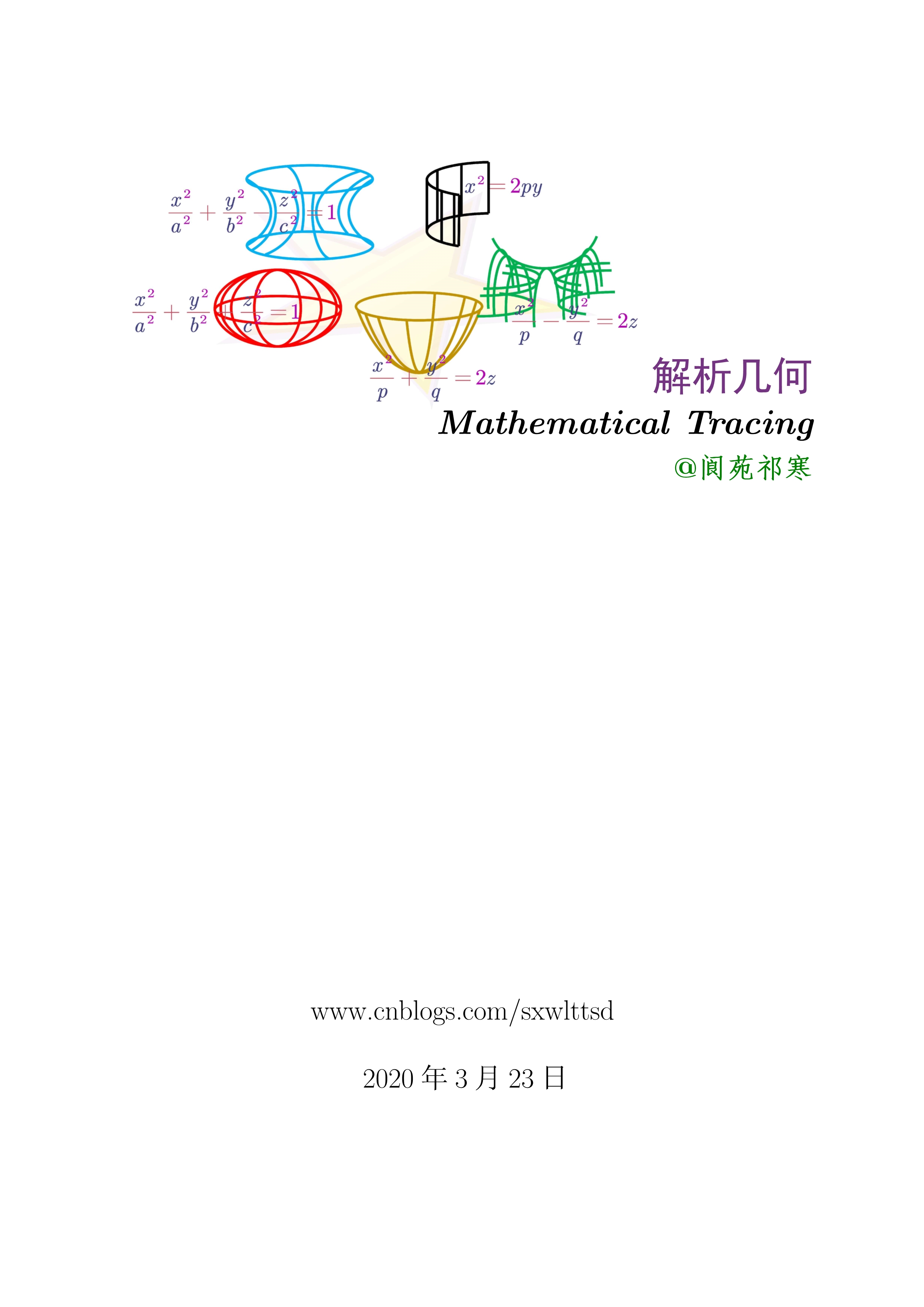page1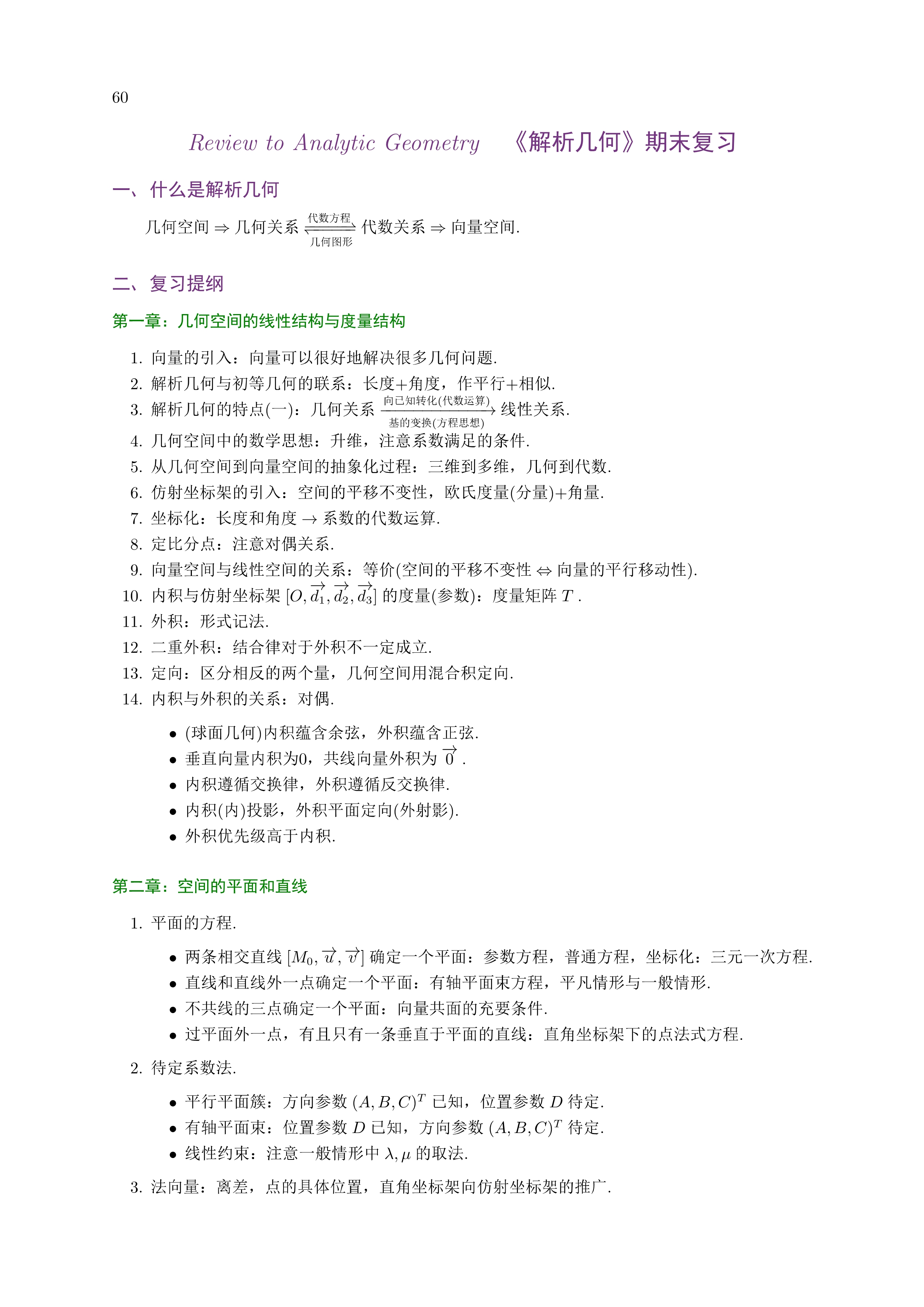page2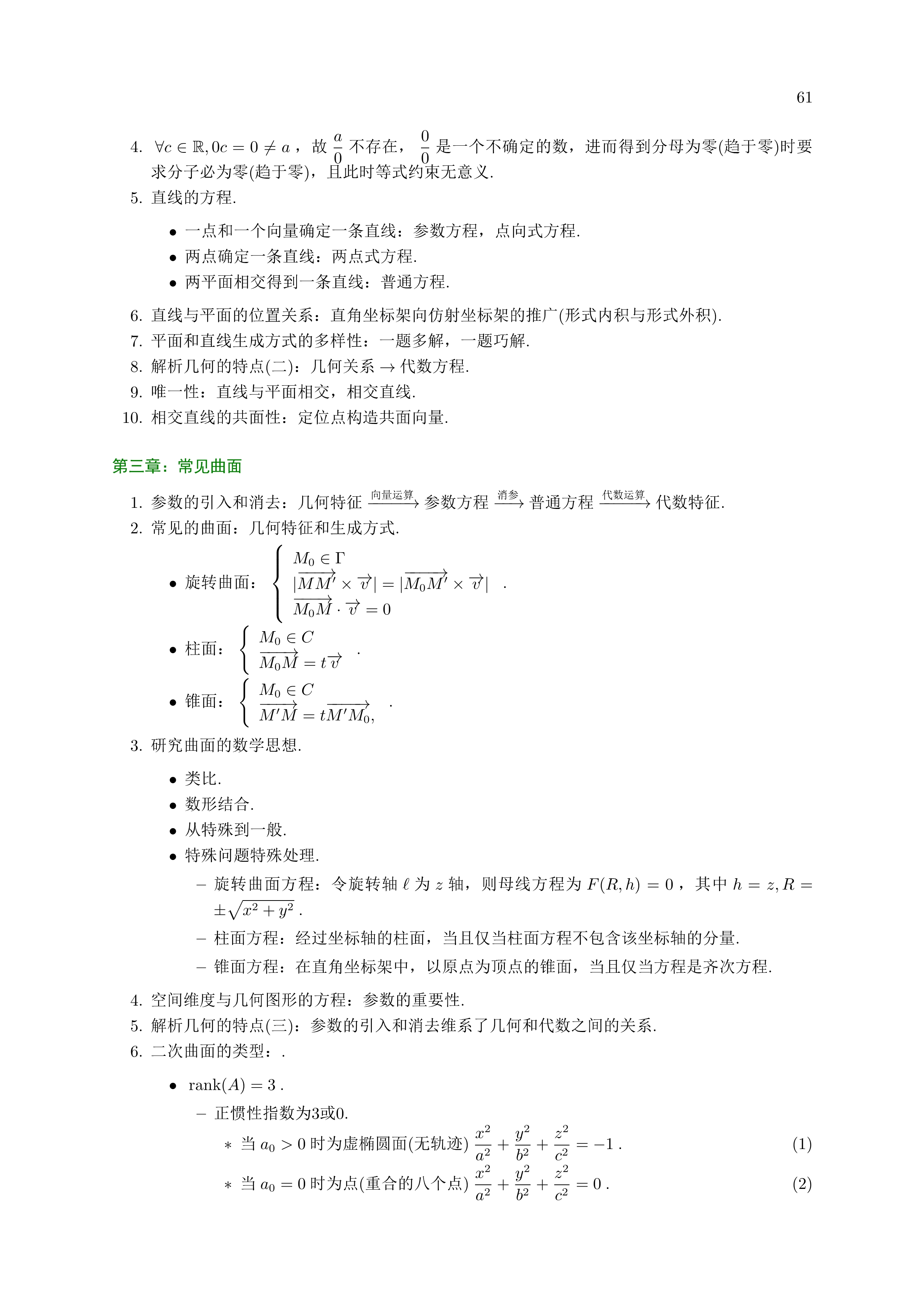page3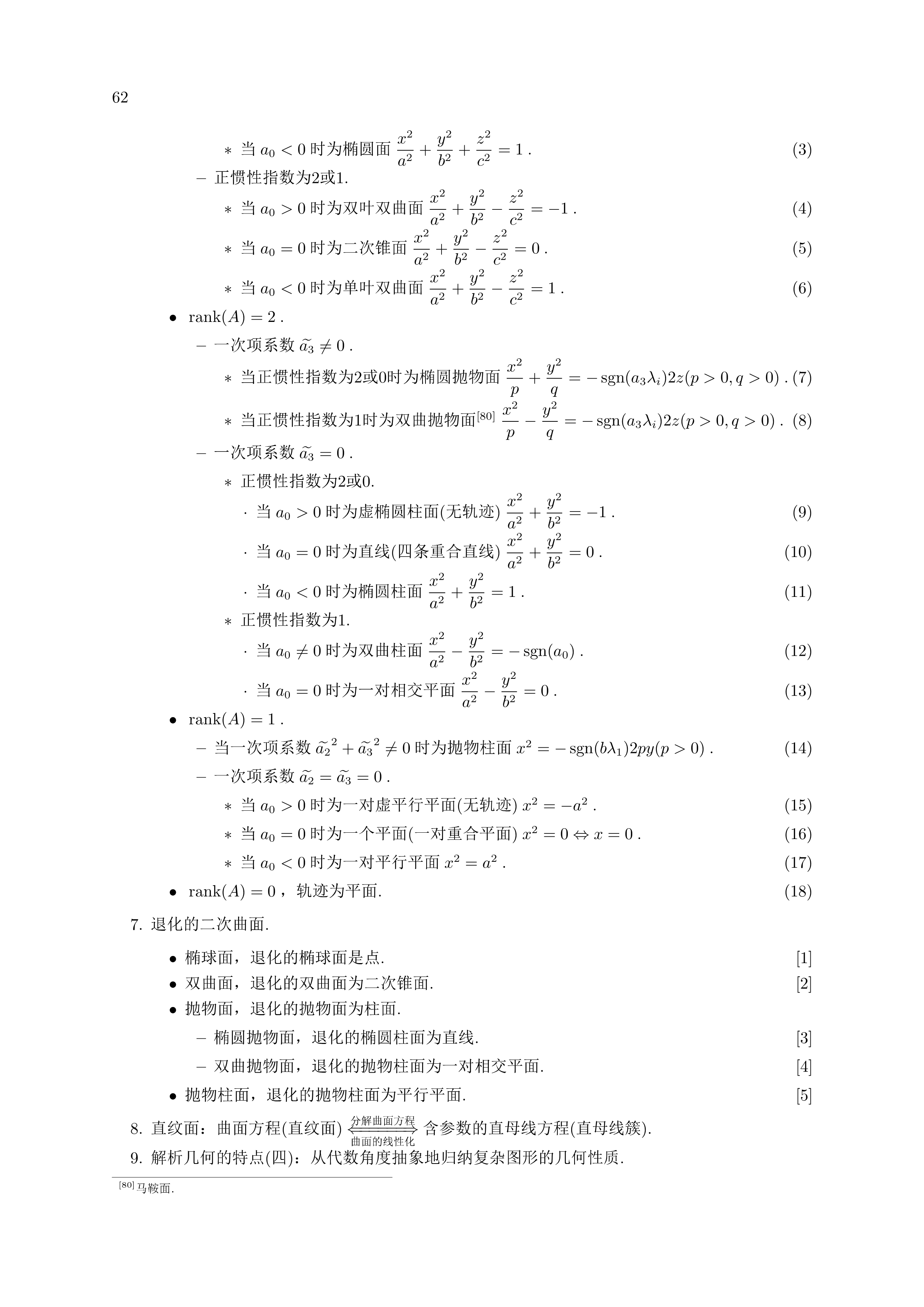page4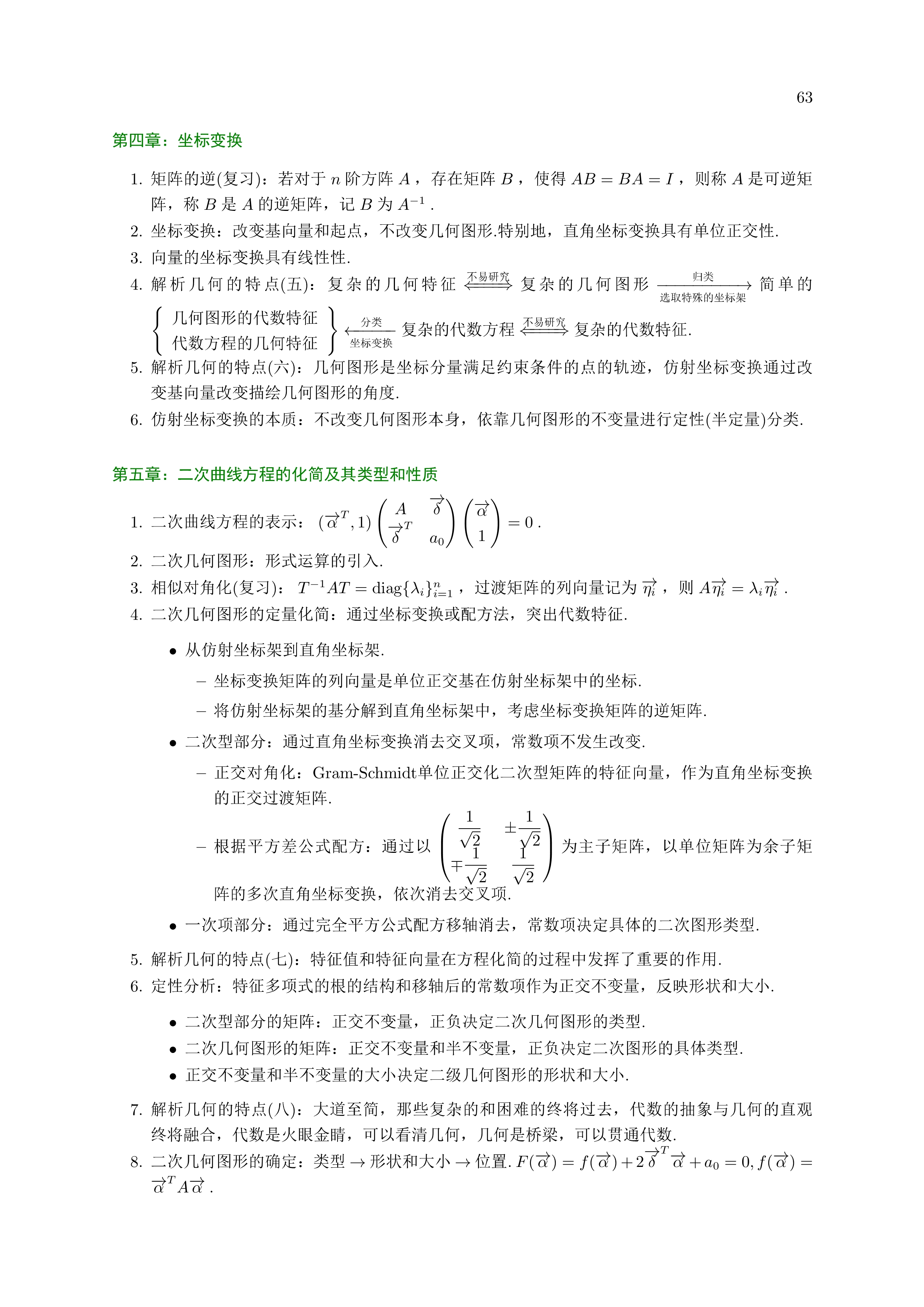page5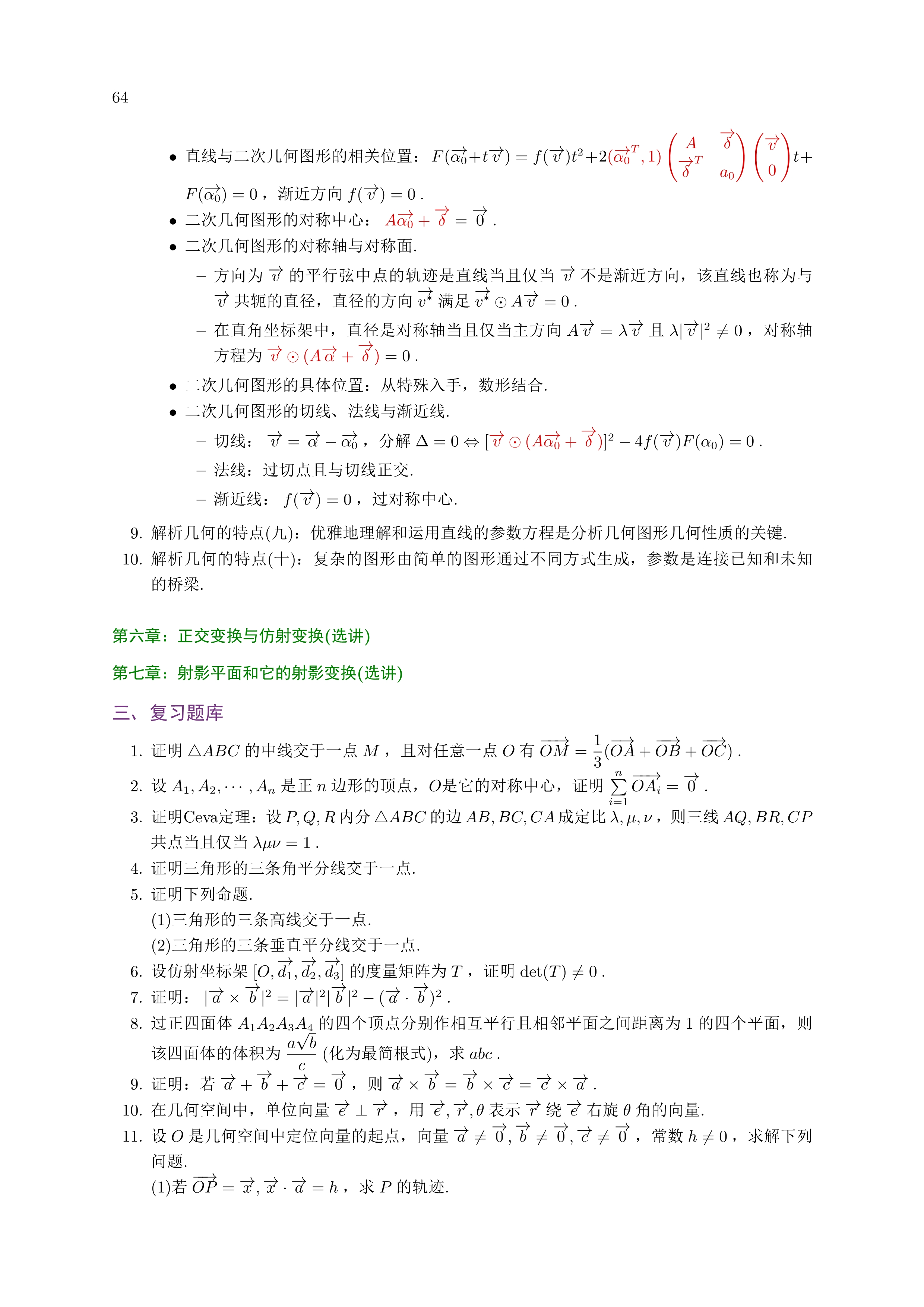page6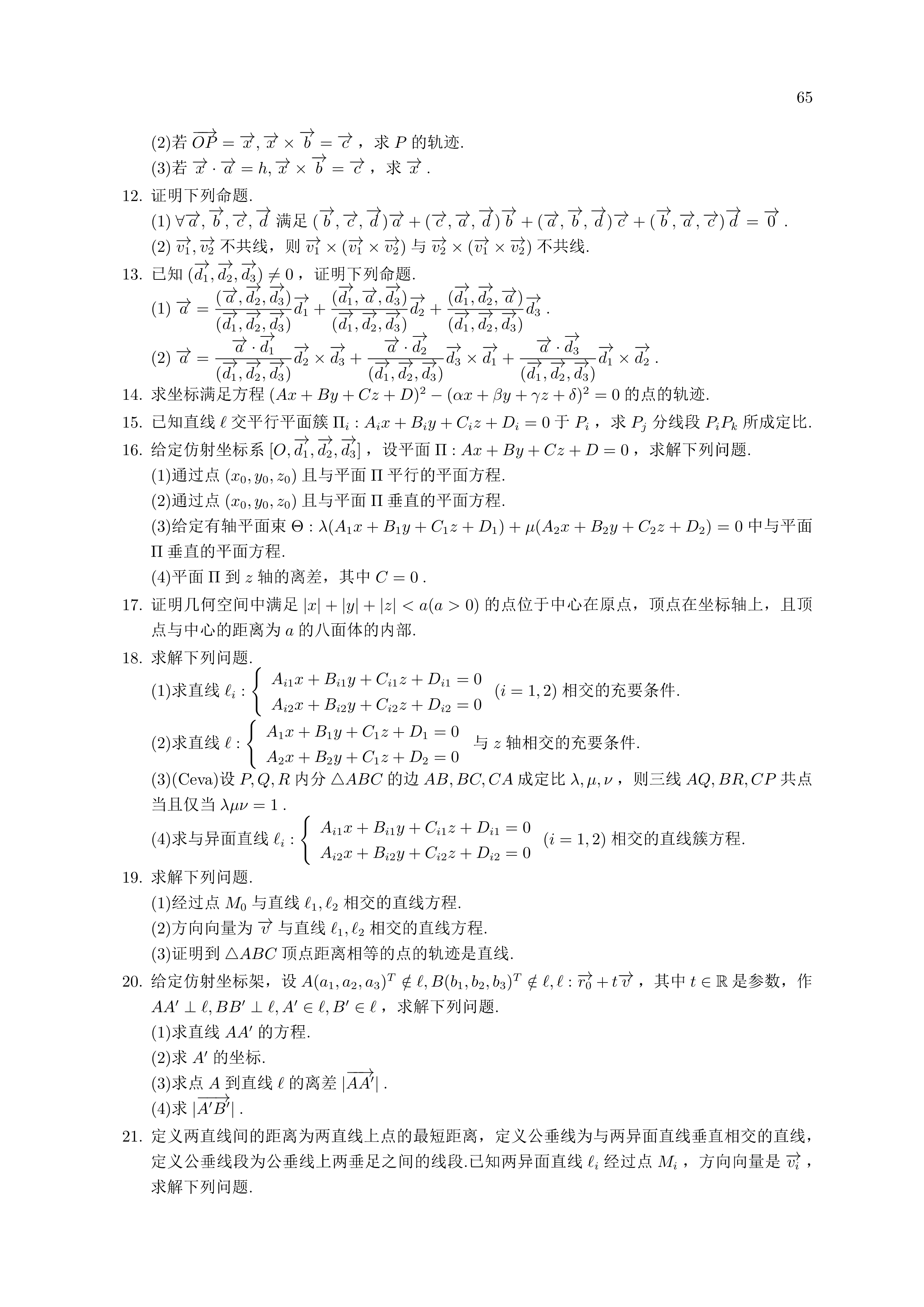page7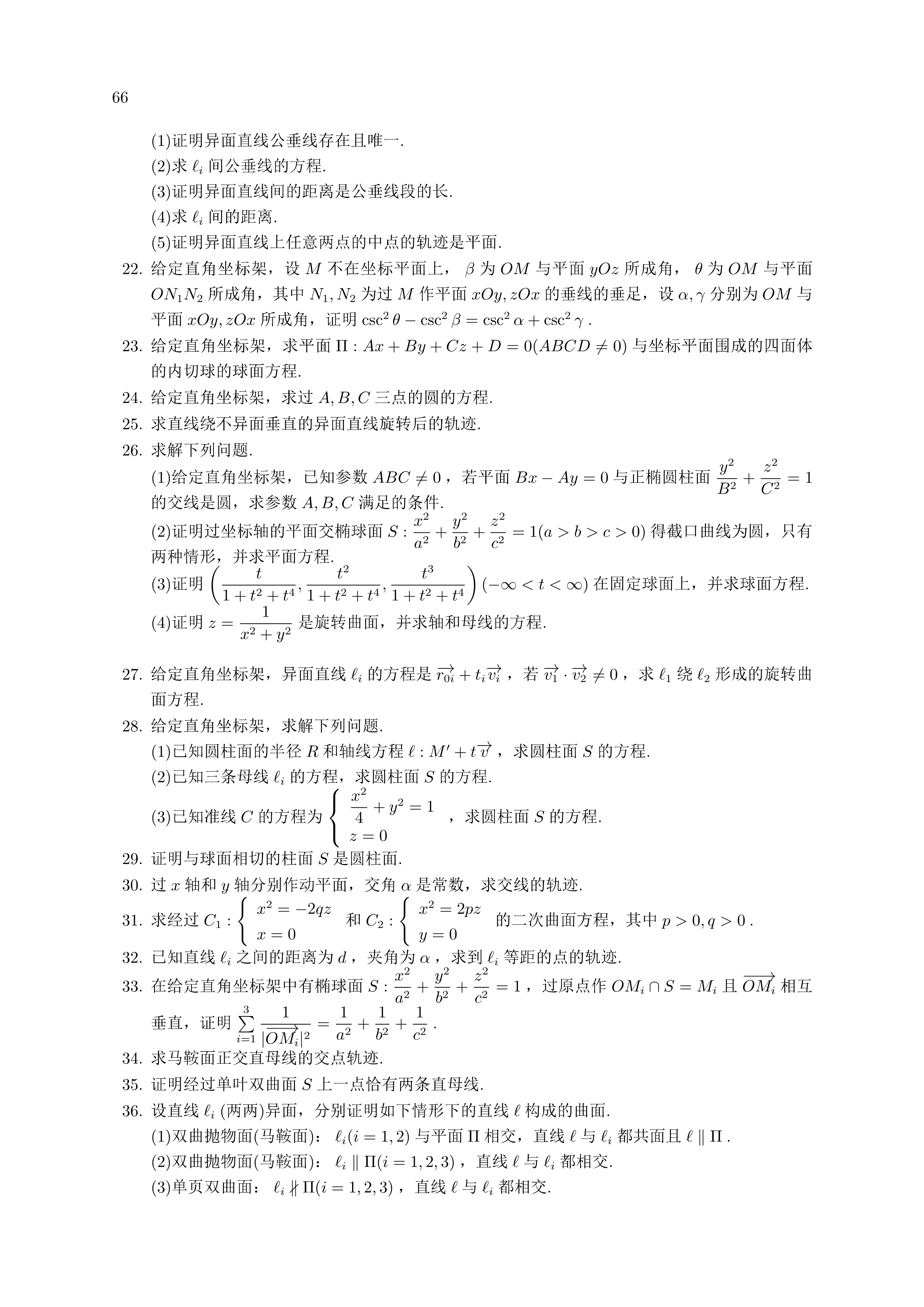page8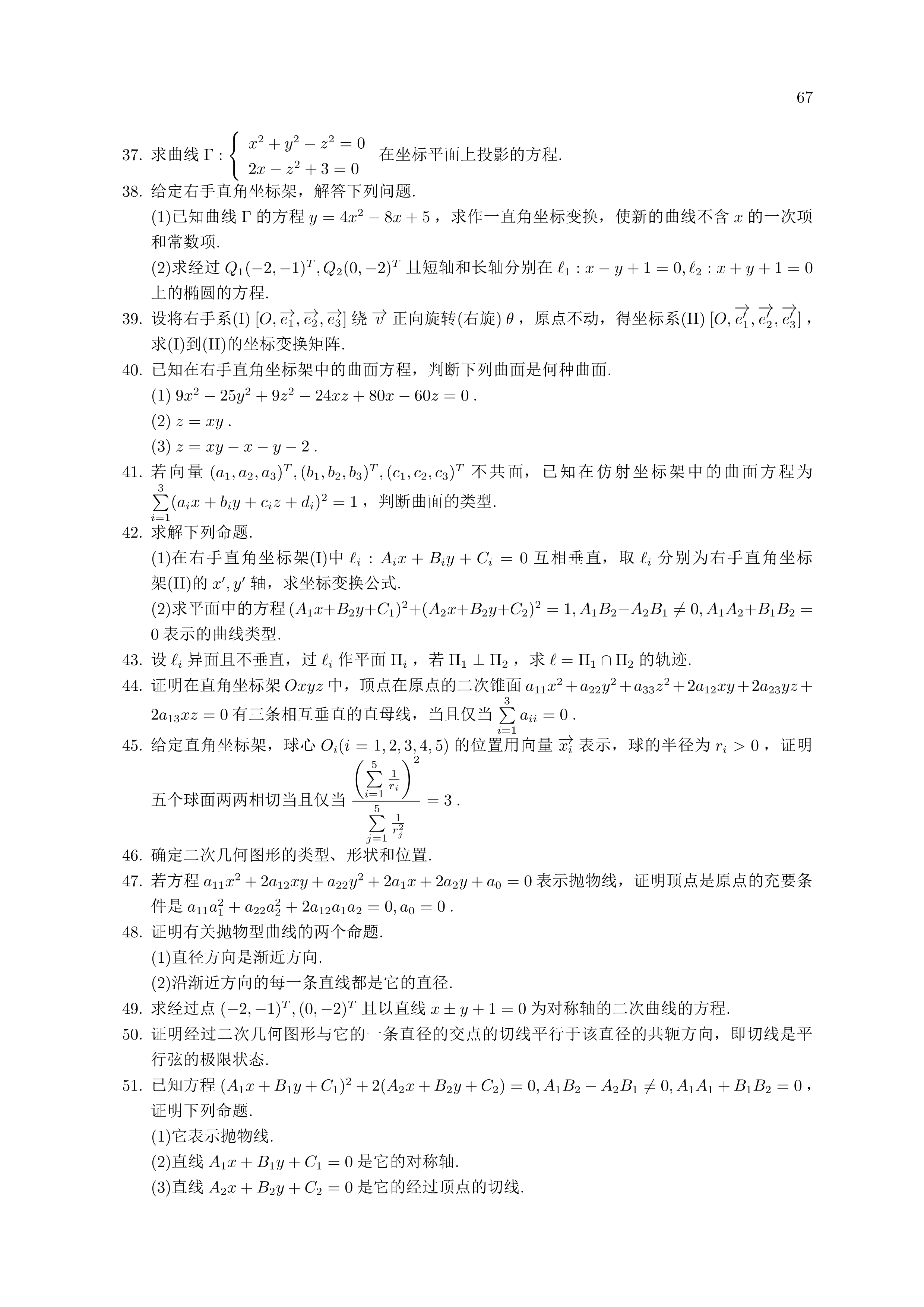page9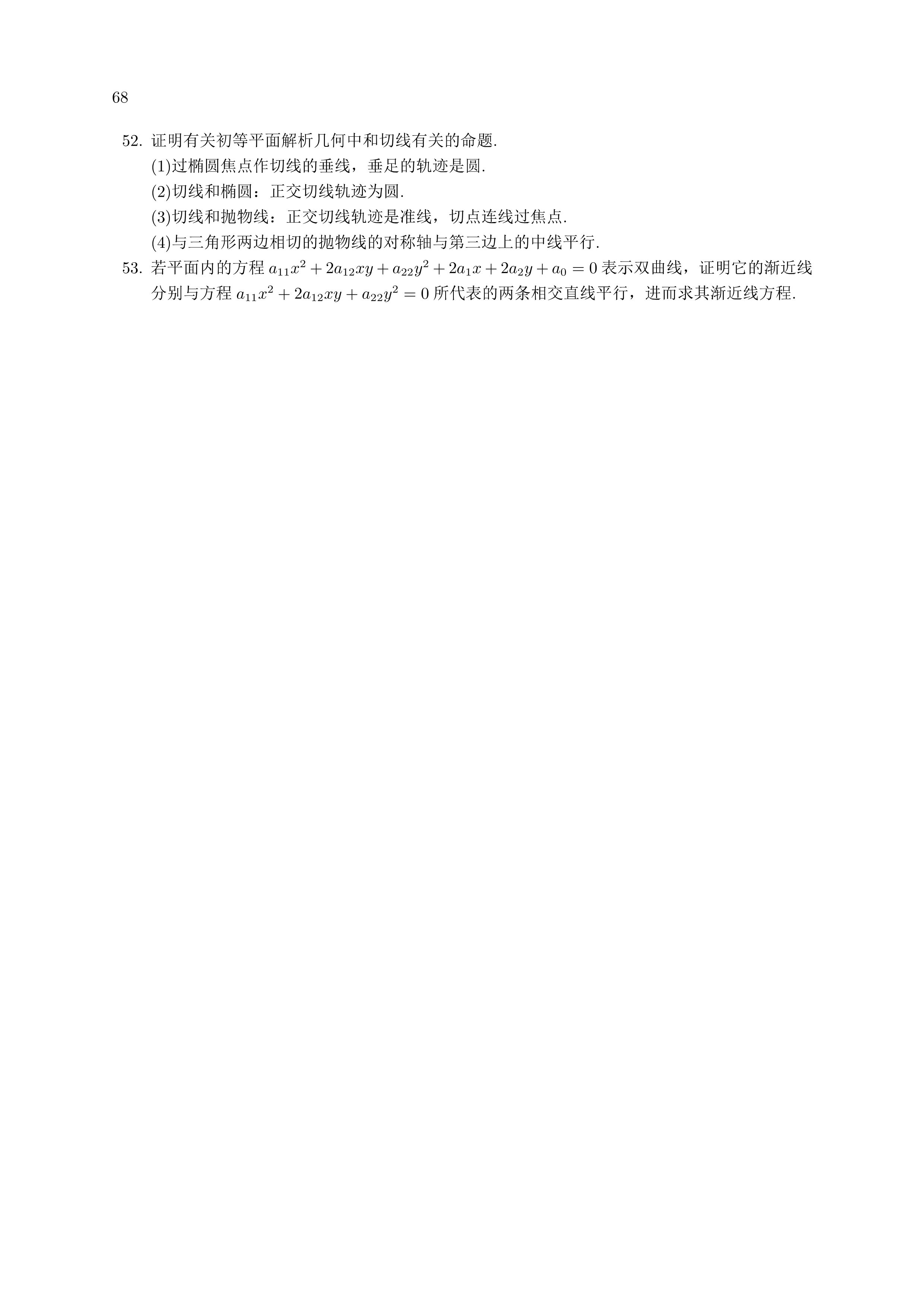exam1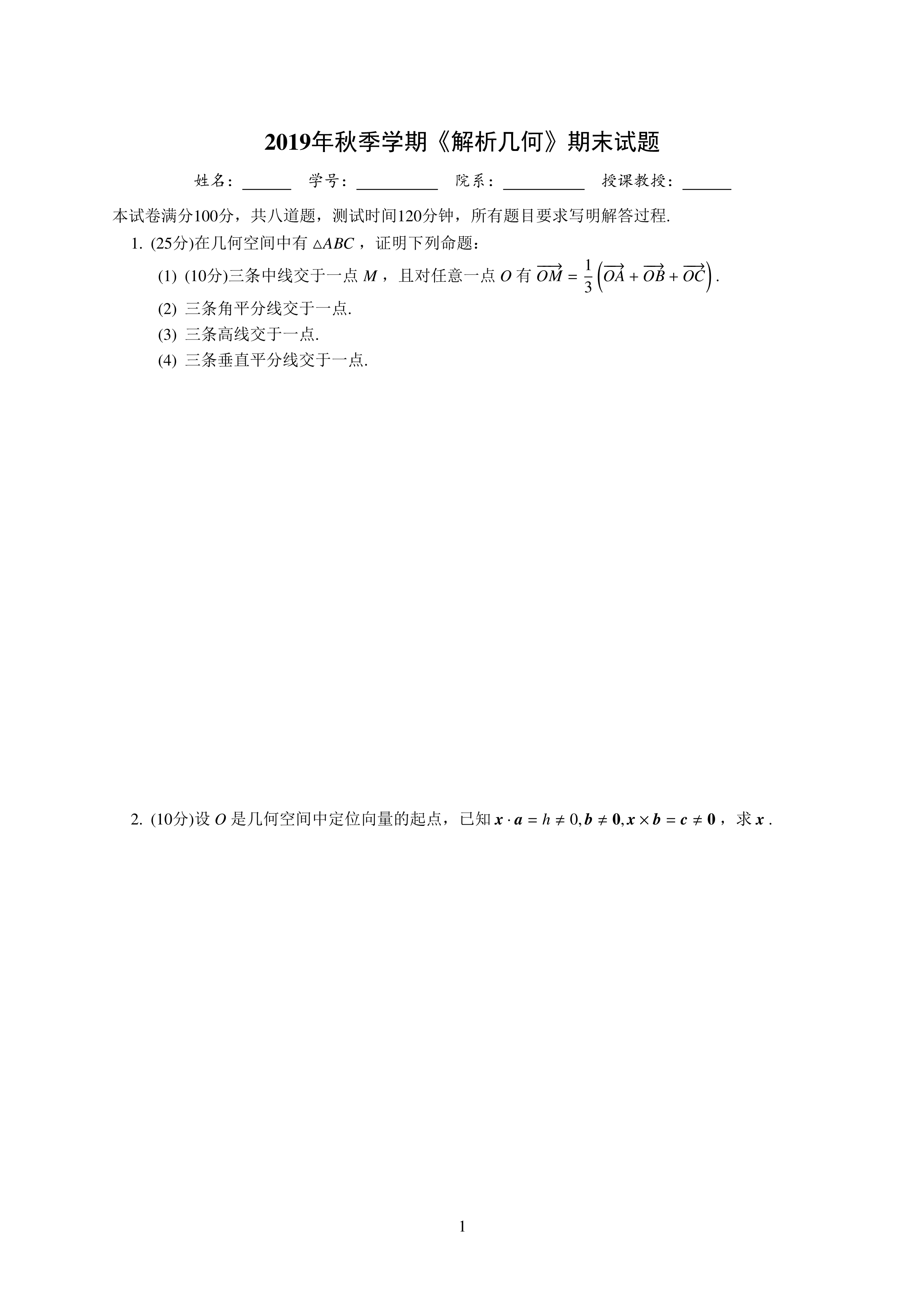exam2exam3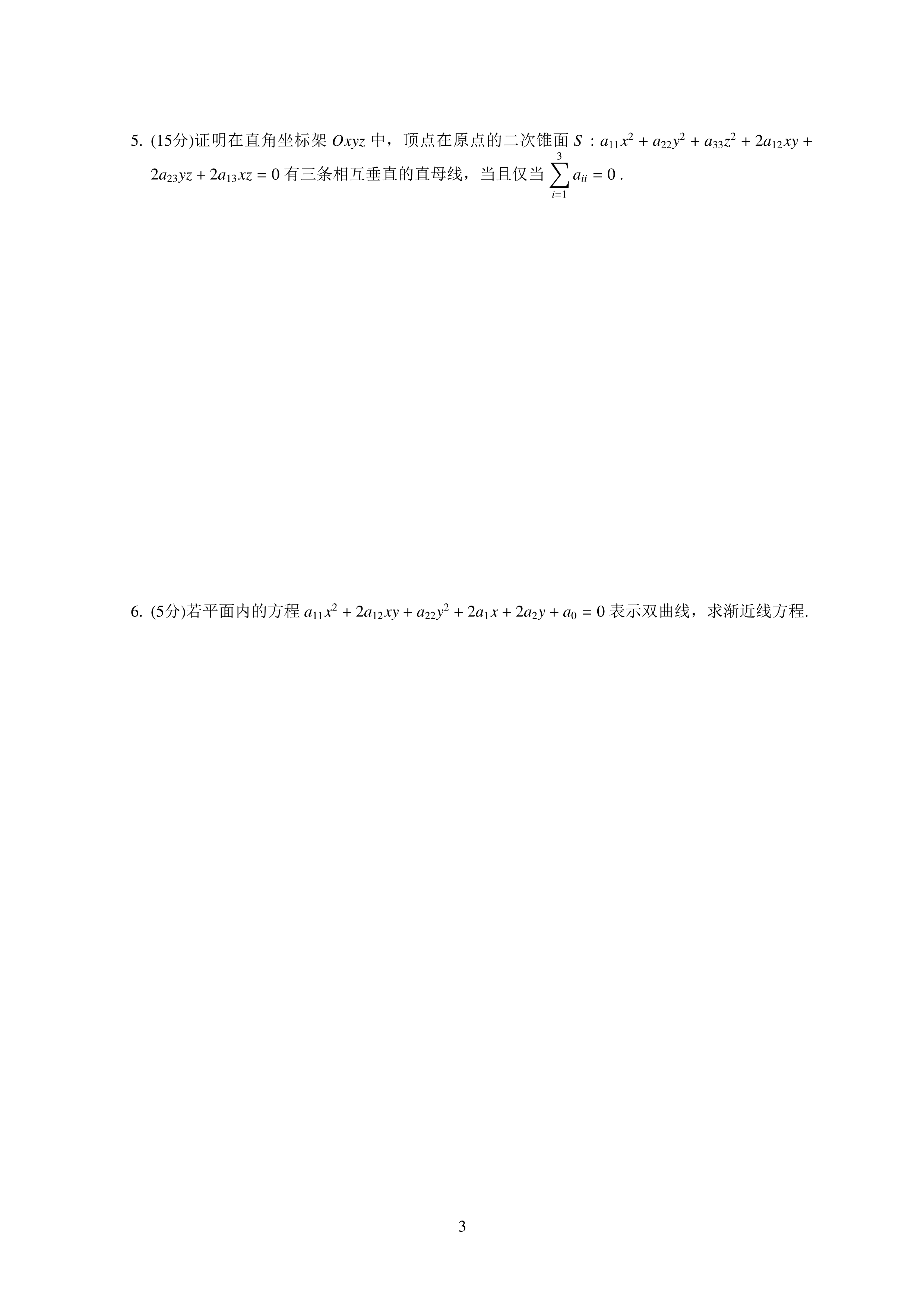exam4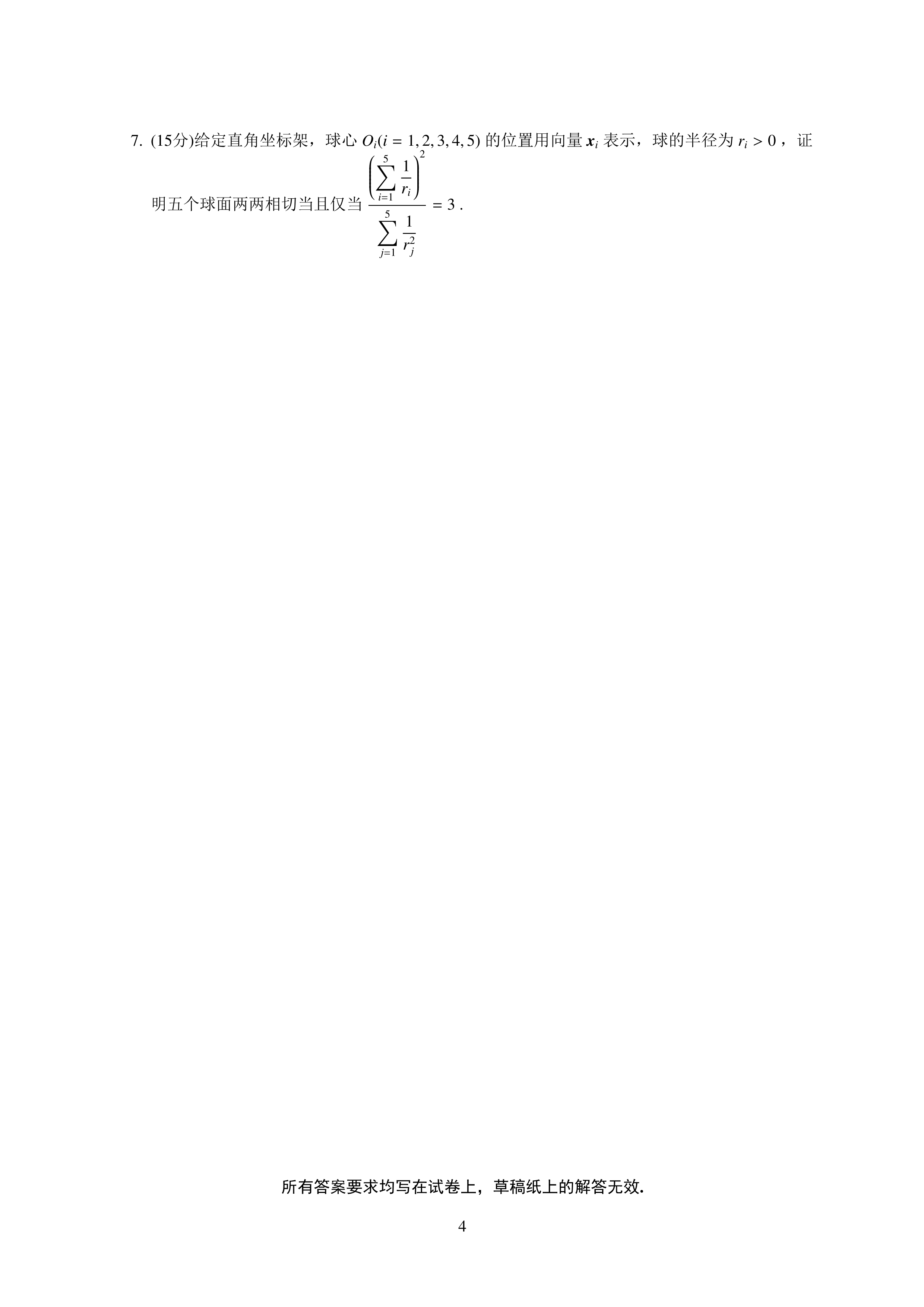ans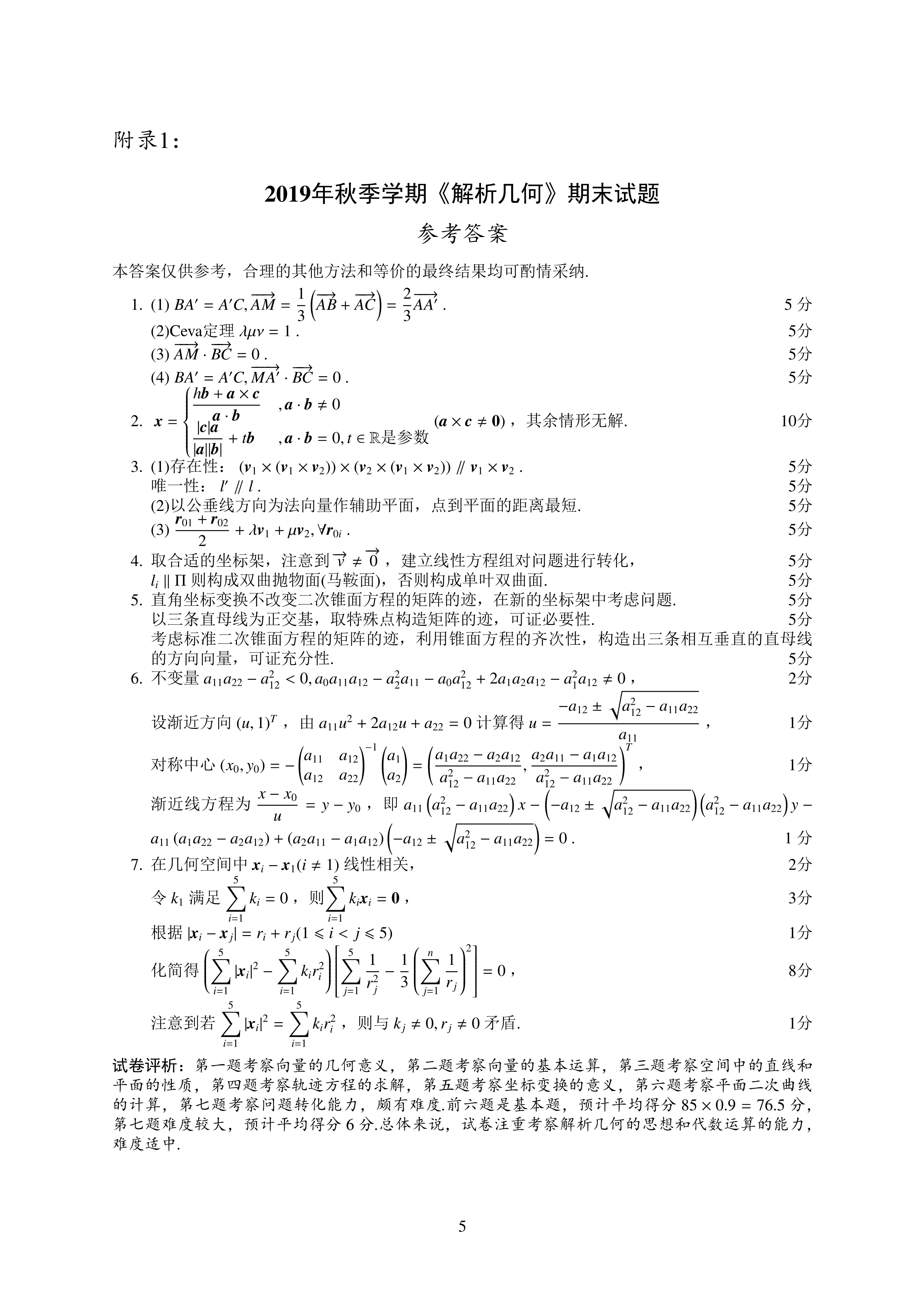postscriptCopyright ©2019-2020 阆苑祁寒
解析几何的更多内容参见：解析几何与整体微分几何
对于部分随笔中图片无法显示的问题，建议使用Ctrl+F5刷新页面，或者更换浏览器。
针对本随笔中图片无法显示的问题，也可以在相册collection1中查看原图，或直接浏览pdf文件。

展开全文• 解析几何解析几何解析几何解析几何解析几何解析几何解析几何解析几何解析几何解析几何
• 第25卷第1期 柳州师专学报 VoL25No1 2010年2月 JournalofLiuzhouTeachers Feb2010 CoUege 吴建生周优军 (柳州师范高等专科学校数学与计算机科学系广西柳州545004) 摘要利用MATLAB辅助解析几何教学借助编程实现解析...
• 但是当时也仅仅是停留在想法上面，因为高中的解析几何虽然步骤程序化，但是有时候需要灵巧的因式分解，感觉以目前的编程水平还是写不出来，但是了解到数学有一个分支——计算几何，专门利用计算机来进行几何计算的...
写在前面：刚学专业课的时候，记得有天突发奇想，心说高三数学的压轴题能不能写个程序跑出答案，这样岂不是解放了数万苦逼高三生的双手？但是当时也仅仅是停留在想法上面，因为高中的解析几何虽然步骤程序化，但是有时候需要灵巧的因式分解，感觉以目前的编程水平还是写不出来，但是了解到数学有一个分支——计算几何，专门利用计算机来进行几何计算的一门科学，并且还与计算机图形学、计算机视觉和图像处理、机器人、计算机辅助设计和制造等高深学科有着联系(摘自《计算几何与应用》导言)，所以今天怀着激动的心情开始了这个专题的学习。数学的一大功用就是计算，而计算的前提便是把自然界中的数据抽象成数学语言，数轴、坐标系都是为了更好的计算而引入的工具，同样，向量——也是这个作用。众所周知，向量有两个必不可少的因素——大小、方向。而在实际问题中，向量即作为有大小、有方向的具有实际物理意义的载体，也是进行代数运算的重要载体，而计算几何中则充分体现了向量的这一特点。我们就一个题目来初涉计算几何这个领域：关于n边形的面积求解的问题。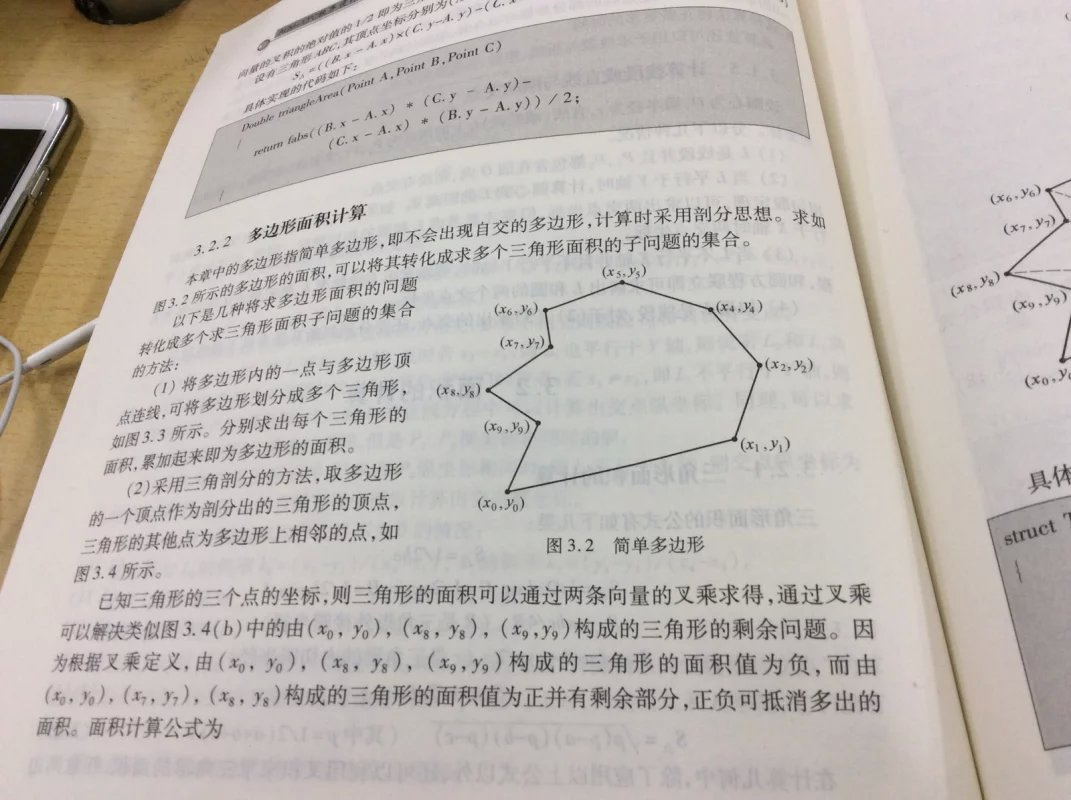如果给出你n边形的n个顶点的坐标，你能求出这个n边形的面积么？如果想要编程实现，显然我们需要让这个求解的过程变得自动化、规律化。我们可以考虑，将其依次分割成多个三角形，然后依次求出处三角形的面积。而如何分割呢？我们考虑，从某个点出发，然后依次连接多边形的两个顶点，然后就可以把图形分割成多个三角形。这个点可以在多边形内部，可以是多边形的顶点，可以某个顶点。像下面这几种分割方法。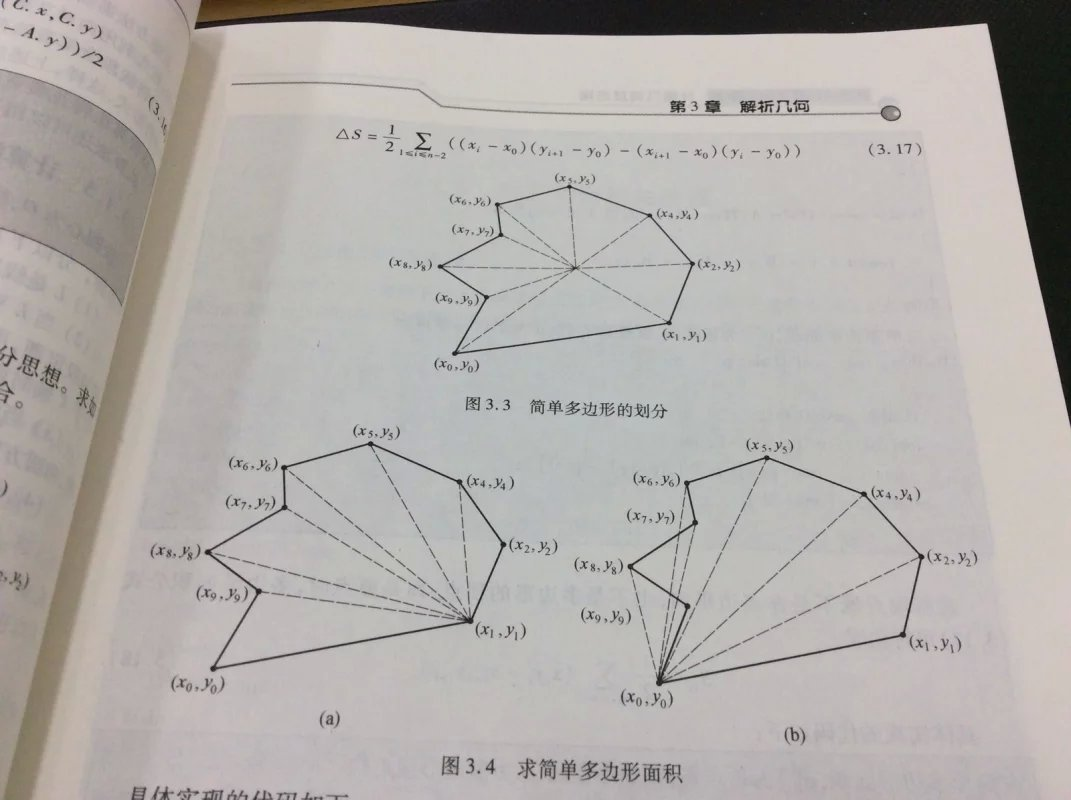我们来看图3.3，显然，现在我们的目标是依次求出所有的三角形然后加和即可，这在编程上也很好实现，但是现在问题是，给出的是点的坐标，我们如何更方便快捷的计算出三角形的面积呢？这里的方法涉及向量叉积的概念了。向量的叉积：我们知道，向量是既有大小又有方向的，对于确定的两个向量a，向量b，通过平移，是可以组成一个确定的三角形的(SAS，其详细证明笔者认为《几何原本》可能会给出)。而我们再根据三角形的面积计算公式s = 1/2*a*b*sinθ(θ是向量a、b的夹角，这里公式不予证明)，以及高中学到的向量的点积公式，我们可以做出如下推导。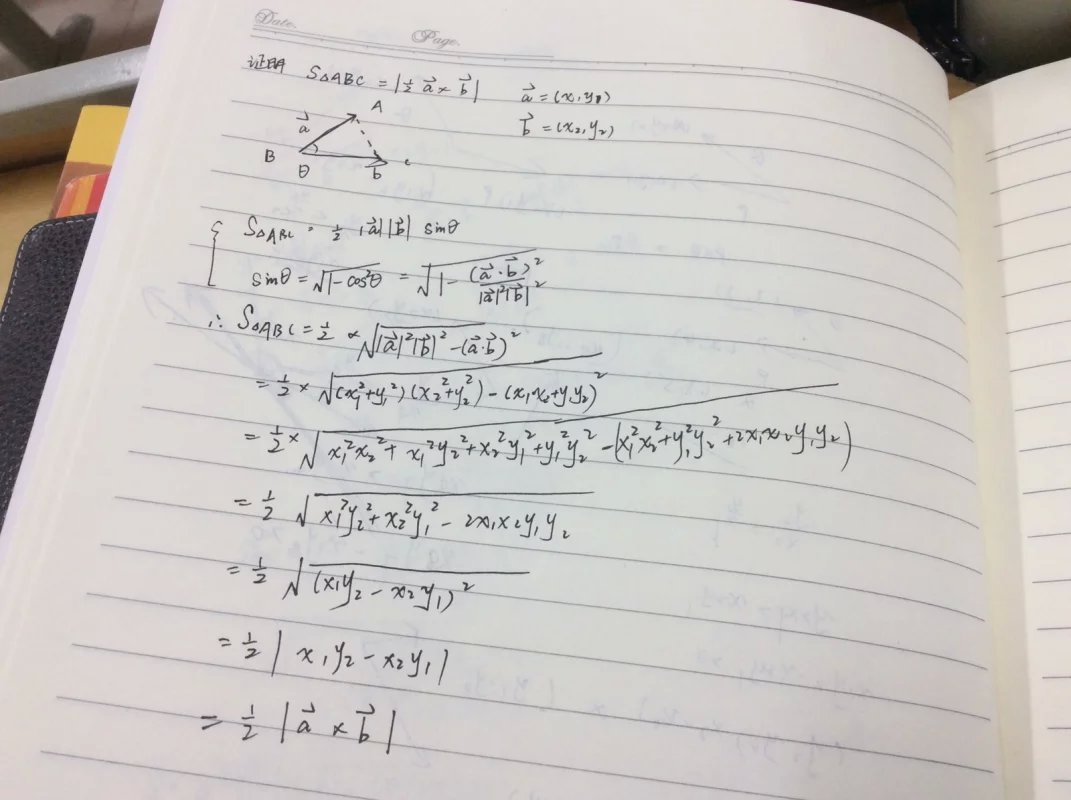那么我们可以得出结论，三角形的面积实际可以通过任意两个边所形成的向量a、b通过叉积运算得来。然而我们看到，如果分割过程没有将n边形分成标准的三角形怎么办？如图3.4(b)，显然上面的方法是存在不足的。从叉积的定义我们可以看出，叉积运算也是有符号的。通过简单的证明，我们可以找到叉积运算的符号规律。(这个探讨好像偏离了问题，但是是对后面探索的一个铺垫)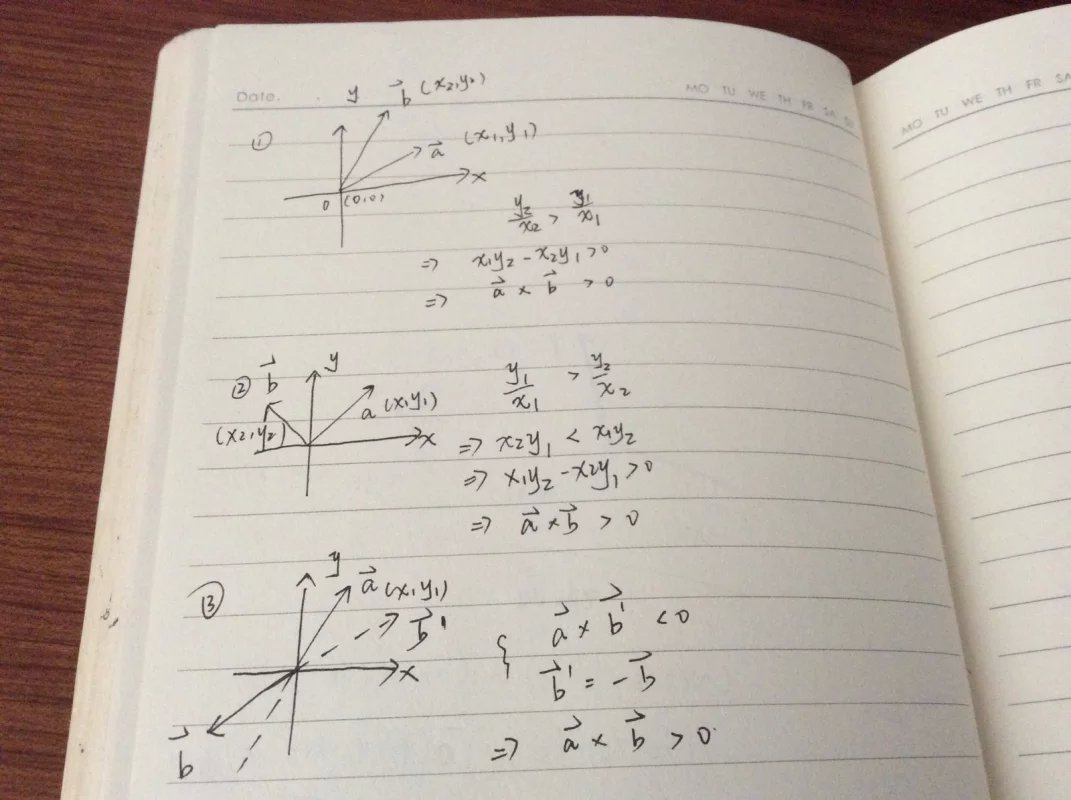我们可以得出这样一个规律——如果向量b的方向在向量a的逆时钟旋转π的范围里，那么向量a×向量b的值是大于零的。我们再回到刚刚的论证当中，显然我们会考虑到，如果这多边形是凹的，就可能出现不完全分割的情况，而在编程过程中我们给出的算法显然要呈现出一般化的规律，因此无法保证能否找到一个合适的点进行分割，所以我们猜测，是否完全分割和不完全分割对于面积的计算是遵循一个公式呢？让我换一个简单的模型来探究一下 这种不完全分割是怎么来的，这里有个误区——认为凹多边形一定会不完全分割，其实不然，图3.4的a、b的对比就佐证了这个观点。这个模型是这样来的，我们规定了AB的斜率不动，然后让顶点o可以随意移动。在情况1中，我们看到，S△AOB被“挤”成了一条直线。在情况2中，AO的斜率是小于AB的，此时可以完全分割，计算面积只需依次就出三角形的面积再加和即可。而S△AOB= 向量AB × 向量OA恰好是正值，这也呼应了前面的过程。在情况3中，情况则大不相同，这里S△AOB一部分是被掏空的，一部分被计算了两次，因此在面积求和的时候应该减掉一个S△AOB，而此时向量AB × 向量OA也恰好是符号，再次呼应，这也为给出不规则多边形面积公式奠定了基础。这是一个最简单的模型，推广以后(精髓所在)，便可以得到上图给出的公式。基于以上的证明，我们来做一道简单的题目来完成算法的代码实现。(Problem source : hdu 2036)Problem Description“ 改革春风吹满地, 不会AC没关系; 实在不行回老家， 还有一亩三分地。 谢谢!(乐队奏乐)”话说部分学生心态极好，每天就知道游戏，这次考试如此简单的题目，也是云里雾里，而且，还竟然来这么几句打油诗。 好呀，老师的责任就是帮你解决问题，既然想种田，那就分你一块。 这块田位于浙江省温州市苍南县灵溪镇林家铺子村，多边形形状的一块地，原本是linle 的，现在就准备送给你了。不过，任何事情都没有那么简单，你必须首先告诉我这块地到底有多少面积，如果回答正确才能真正得到这块地。 发愁了吧？就是要让你知道，种地也是需要AC知识的！以后还是好好练吧...Input输入数据包含多个测试实例，每个测试实例占一行，每行的开始是一个整数n(3<=n<=100)，它表示多边形的边数(当然也是顶点数)，然后是按照逆时针顺序给出的n个顶点的坐标(x1, y1, x2, y2... xn, yn),为了简化问题，这里的所有坐标都用整数表示。 输入数据中所有的整数都在32位整数范围内，n=0表示数据的结束，不做处理。Output对于每个测试实例，请输出对应的多边形面积，结果精确到小数点后一位小数。 每个实例的输出占一行。很明显的多边形求面积的问题，在编程实现的时候，基于上文计算公式的得出，为了方便计算，我们取原点为分割凸多边形的那个点，这样我们要遍历到多边形的n个顶点，并通过叉积来计算小三角形的面积。遍历到第n个顶点的时候，第n个顶点会和第1个顶点构成三角形，因此我们需要将记录点集的数组的末尾再添加一个元素——即第1个顶点，这才计算几何问题中遍历点集进行运算是很常见的操作。参考代码如下。#include#includeusing namespacestd;structpoint{doublex,y;} P;doublecross(point a,point b){return a.x*b.y-a.y*b.x;}double area(intn){double tmp=0;for(int i=0;i{tmp+=cross(P[i],P[i+1]);}return tmp/2.00;}intmain(){inti,n;while(scanf("%d",&n),n){for(i=0;iscanf("%lf%lf",&P[i].x,&P[i].y);P[n].x=P.x;P[n].y=P.y;printf("%.1lf\n",area(n));}return 0;}有关三角形和圆的结合。(Problem source : 1374)Problem DescriptionTo calculate the circumference of a circle seems to be an easy task - provided you know its diameter. But what if you don't?You are given the cartesian coordinates of three non-collinear points in the plane. Your job is to calculate the circumference of the unique circle that intersects all three points.InputThe input file will contain one or more test cases. Each test case consists of one line containing six real numbers x1,y1, x2,y2,x3,y3, representing the coordinates of the three points. The diameter of the circle determined by the three points will never exceed a million. Input is terminated by end of file.OutputFor each test case, print one line containing one real number telling the circumference of the circle determined by the three points. The circumference is to be printed accurately rounded to two decimals. The value of pi is approximately 3.141592653589793.题目大意，让你给出三角形的三个顶点，然后让你计算出该三角形的外接圆的周长。这里我们需要推导一下计算外接圆半径的公式。根据正弦定理我们知道，c/sinc =2r，而s = 1/2a*b*sinc，我们可以得到r =a*b*c / 4s。这里求三角形的面积s的时候，我们常用s = sqrt(p*(p-a)*(p-b)*(p-c))这个公式，其中p = 0.5(a + b + c)。有了明确的公式，算法是实现上非常容易。代码如下。#include#include#define PI 3.141592653589793typedefstructpoint{doublex ,  y;}Point;doubledistance(Point a ,Point b){return sqrt((a.x - b.x)*(a.x - b.x) + (a.y - b.y)*(a.y -b.y));}intmain(){doublea , b , c , p  , r , s , c_of_circle;Point v;while(scanf("%lf %lf %lf %lf %lf %lf",&v.x , &v.y , &v.x ,&v.y , &v.x , &v.y) !=EOF){a= distance(v , v);b= distance(v , v);c= distance(v , v);p= (a + b + c)/2.0;s= sqrt(p * ( p - a ) * ( p - b ) * ( p -c ));r= (a*b*c) / (4.0*s);c_of_circle= 2*PI*r;printf("%.2lf\n",c_of_circle);}}我们再来看一道关于求解三角形外接圆的题目。(Problem soure : poj 1329)DescriptionYour team is to write a program that, given the Cartesian coordinates of three points on a plane, will find the equation of the circle through them all. The three points will not be on a straight line.  The solution is to be printed as an equation of the form(x - h)^2 + (y - k)^2 = r^2(1)and an equation of the formx^2 + y^2 + cx + dy - e = 0(2)InputEach line of input to your program will contain the x and y coordinates of three points, in the order Ax, Ay, Bx, By, Cx, Cy. These coordinates will be real numbers separated from each other by one or more spaces.OutputYour program must print the required equations on two lines using the format given in the sample below. Your computed values for h, k, r, c, d, and e in Equations 1 and 2 above are to be printed with three digits after the decimal point. Plus and minus signs in the equations should be changed as needed to avoid multiple signs before a number. Plus, minus, and equal signs must be separated from the adjacent characters by a single space on each side. No other spaces are to appear in the equations. Print a single blank line after each equation pair.题目大意：基础三个点的坐标，让你输出对应外接圆的顶点式和一般式。数理分析：这里我们假设给出的三点坐标为(x1,y1)，(x2,y3)，(x3,y3)根据外心的定义，我们需要找到两条中垂线的交点。拿出两个点p1(x1,y1)，p2(x2,y2)来说，中垂线过p1p2的中点，并且斜率与p1p2所成直线的斜率的乘积是-1，通过简单的解析几何的知识，我们可得到一条中垂线的参数方程。(x-(x1+x2)/2)(x1-x2) = -(y1-y2)(y-(y1+y2)/2)。同理再得到一条这样的方程，两个方程进行连理，既可以一个三角形外心通用的计算公式。知道了圆心的坐标，我们只需计算圆心到某个顶点的距离，即可得到半径r。编程实现：题目给出的格式比较繁琐，这里注意分情况讨论一下处理一下符号即可。参考代码如下。#include#includeusing namespacestd;structpoint{doublex , y;}p,o;doubledis(point a , point b){return sqrt((a.x-b.x)*(a.x-b.x) + (a.y-b.y)*(a.y-b.y));}void get_C(point p1,point p2,point p3)//求圆心{doublet1,t2,t3;t1=p1.x*p1.x+p1.y*p1.y-p2.x*p2.x-p2.y*p2.y;t2=p1.x*p1.x+p1.y*p1.y-p3.x*p3.x-p3.y*p3.y;t3=2*(p1.x-p2.x)*(p1.y-p3.y)-2*(p1.x-p3.x)*(p1.y-p2.y);o.x=((p1.y-p3.y)*t1-(p1.y-p2.y)*t2)/t3;o.y=-((p1.x-p3.x)*t1-(p1.x-p2.x)*t2)/t3;}intmain(){while(scanf("%lf %lf %lf %lf %lf %lf",&p.x , &p.y , &p.x ,&p.y , &p.x , &p.y) !=EOF){get_C(p,p,p);double r = dis(p,o);double t=r*r-o.x*o.x-o.y*o.y;if(o.x<0&&o.y<0){printf("(x + %.3lf)^2 + (y + %.3lf)^2 = %.3lf^2\n",-o.x,-o.y,r);if(t>=0)printf("x^2 + y^2 + %.3lfx + %.3lfy - %.3lf = 0\n",-2*o.x,-2*o.y,t);elseprintf("x^2 + y^2 + %.3lfx + %.3lfy + %.3lf = 0\n",-2*o.x,-2*o.y,-t);}else if(o.x<0){printf("(x + %.3lf)^2 + (y - %.3lf)^2 = %.3lf^2\n",-o.x,o.y,r);if(t>=0)printf("x^2 + y^2 + %.3lfx - %.3lfy - %.3lf = 0\n",-2*o.x,2*o.y,t);elseprintf("x^2 + y^2 + %.3lfx - %.3lfy + %.3lf = 0\n",-2*o.x,2*o.y,-t);}else if(o.y<0){printf("(x - %.3lf)^2 + (y + %.3lf)^2 = %.3lf^2\n",o.x,-o.y,r);if(t>=0)printf("x^2 + y^2 - %.3lfx + %.3lfy - %.3lf = 0\n",2*o.x,-2*o.y,t);elseprintf("x^2 + y^2 - %.3lfx + %.3lfy + %.3lf = 0\n",2*o.x,-2*o.y,-t);}else{printf("(x - %.3lf)^2 + (y - %.3lf)^2 = %.3lf^2\n",o.x,o.y,r);if(t>=0)printf("x^2 + y^2 - %.3lfx - %.3lfy - %.3lf = 0\n",2*o.x,2*o.y,t);elseprintf("x^2 + y^2 - %.3lfx - %.3lfy + %.3lf = 0\n",2*o.x,2*o.y,-t);}printf("\n");}}我们再来看一些关于最小面积覆盖的问题。(Problem source : hdu 1859)Problem Description给定一系列2维平面点的坐标(x, y)，其中x和y均为整数，要求用一个最小的长方形框将所有点框在内。长方形框的边分别平行于x和y坐标轴，点落在边上也算是被框在内。Input测试输入包含若干测试用例，每个测试用例由一系列坐标组成，每对坐标占一行，其中|x|和|y|小于 231；一对0 坐标标志着一个测试用例的结束。注意(0, 0)不作为任何一个测试用例里面的点。一个没有点的测试用例标志着整个输入的结束。Output对每个测试用例，在1行内输出2对整数，其间用一个空格隔开。第1对整数是长方形框左下角的坐标，第2对整数是长方形框右上角的坐标。读完题后其实不难发现，这道题目的本质其实就是找到这些点的最大的横坐标和纵坐标(右上角的点)，和最小的和坐标和纵坐标(左下角的点)，编程实现上也基本没有什么难度。这道题似乎有点简答的弱智了，然是在简短的代码中其实还是体现了计算几何问题在编程实现时代码的规整之美，计算几何其实就是基于计算机自动化、程序化的特点来解决一些繁琐计算的几何问题，而自动化的背后往往呈现出简单的原理，掌握到背后简单的原理，解决计算几何问题是将会更加游刃有余。由于其实规整的矩形覆盖，所以问题非常好解决，但是如果是圆形呢？那其实就是计算几何里面的一类问题——最小圆覆盖。在后面的文章中我们将继续详细的介绍。参考代码如下:#includeusing namespacestd;intmain(){intmaxx , maxy , minx , miny;intx , y;while(scanf("%d %d" , &x , &y) !=EOF){if(x == 0 && y == 0)  break;maxx= x , minx = x , miny = y , maxy =y;while(1){scanf("%d %d" , &x , &y);if(x == 0 && y == 0){printf("%d %d %d %d\n", minx , miny , maxx , maxy);break;}if(x > maxx) maxx =x;if(x < minx) minx =x;if(y > maxy) maxy =y;if(y < miny) miny =y;}}}我们再来看一道平面解析几何中最基础的问题，关于点的对称、直线交点的计算。(Problem source : hdu2857)Problem DescriptionThe light travels in a straight line and always goes in the minimal path between two points, are the basic laws of optics.Now, our problem is that, if a branch of light goes into a large and infinite mirror, of course，it will reflect, and leave away the mirror in another direction. Giving you the position of mirror and the two points the light goes in before and after the reflection, calculate the reflection point of the light on the mirror.    You can assume the mirror is a straight line and the given two points can’t be on the different sizes of the mirror.InputThe first line is the number of test case t(t<=100).    The following every four lines are as follow:   X1 Y1   X2 Y2   Xs Ys   Xe Ye(X1,Y1),(X2,Y2) mean the different points on the mirror, and (Xs,Ys) means the point the light travel in before the reflection, and (Xe,Ye) is the point the light go after the reflection.The eight real number all are rounded to three digits after the decimal point, and the absolute values are no larger than 10000.0.题目大意：这里先给出两个点得到一条直线，当做镜子。然后给定入射点和出射点，让你计算出这条光线与镜子的交点。数理分析：很基础的几何题目，只需做出入射点关于镜子的对称点，对称点和出射点构成的直线与镜子的交点便是所求作的点。编程实现：数理思维虽然不难，但是体现到编程上还是需要解决很多问题的。就比如：①给定直线的两点，我们如何得到一条直线？②给定两条直线，我们又如何求其交点？③这里的对称点怎么找？这些问题虽然不难，但是还是需要比较巧的推理技巧。关于给定两点求直线，这里我们借助解析几何中常见的斜截式y = kx + b，然后将其转化成一般式ax + by+ c = 0，简单的推导如下。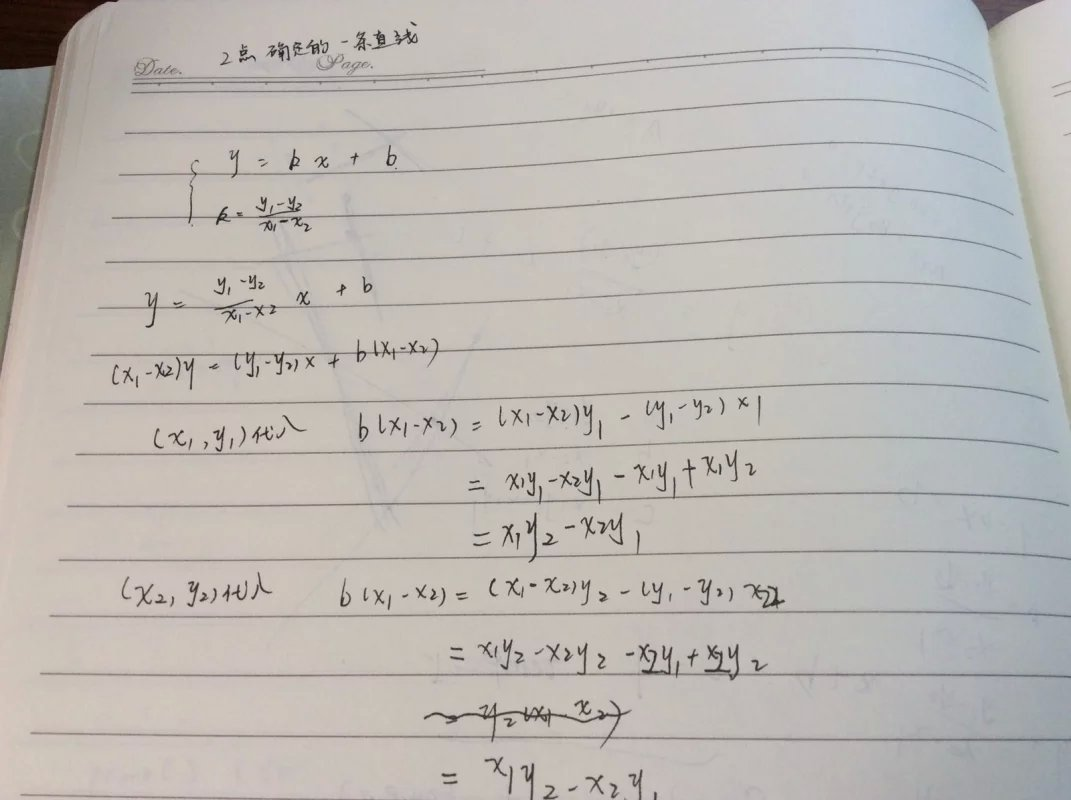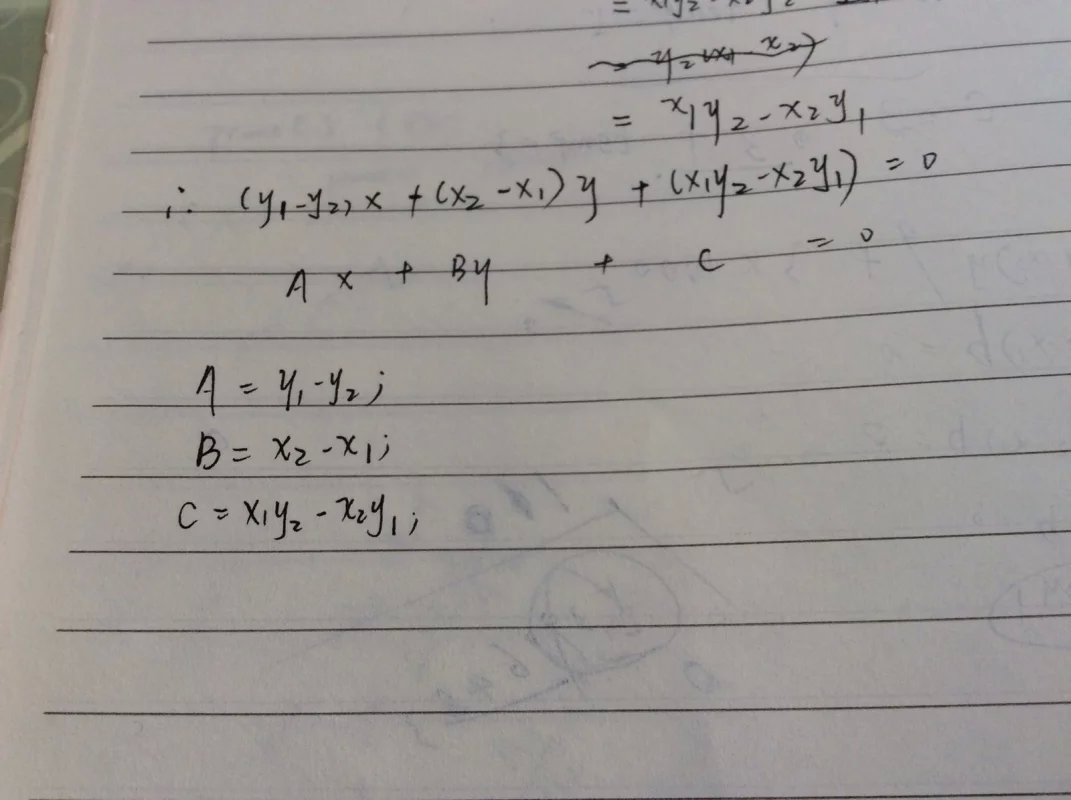关于给定两条直求其交点，我们采取一样的方法。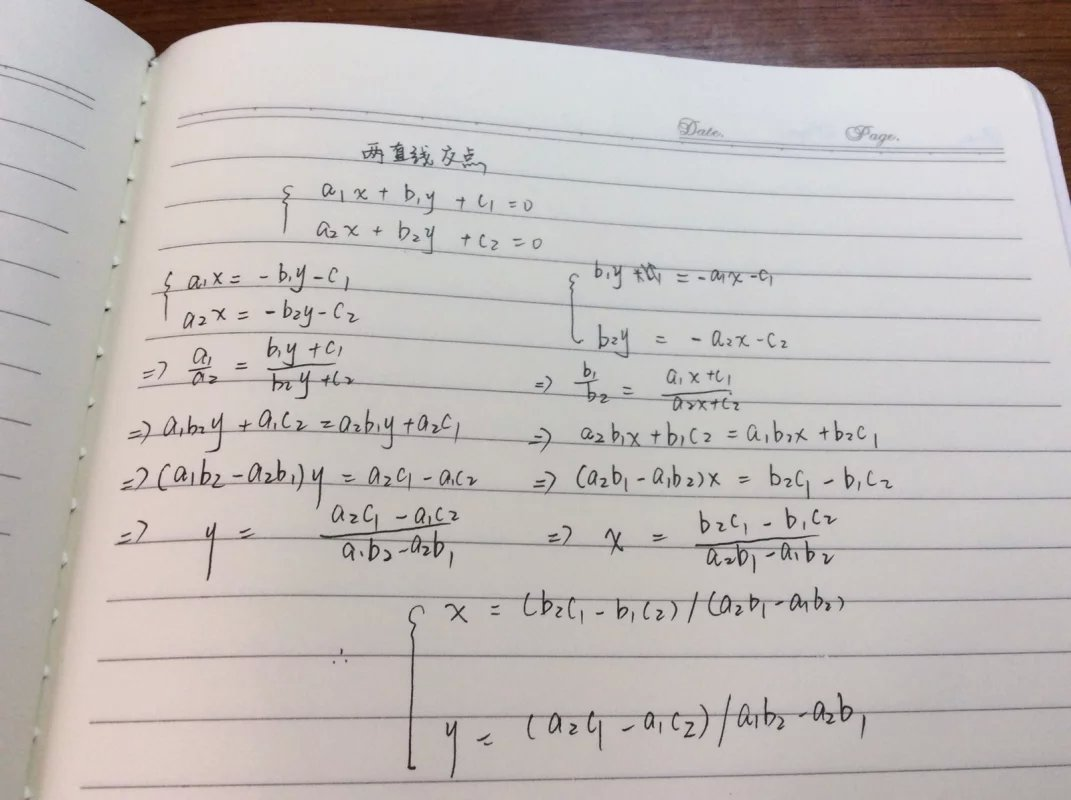而对于找对称点，我们先找到互为对称点的这两个点组成的线段的中点，然后再进行计算。而这个中点恰好又是过入射点且垂直于镜子的直线与镜子的交点，这里的计算也很简单。简单的推导如下。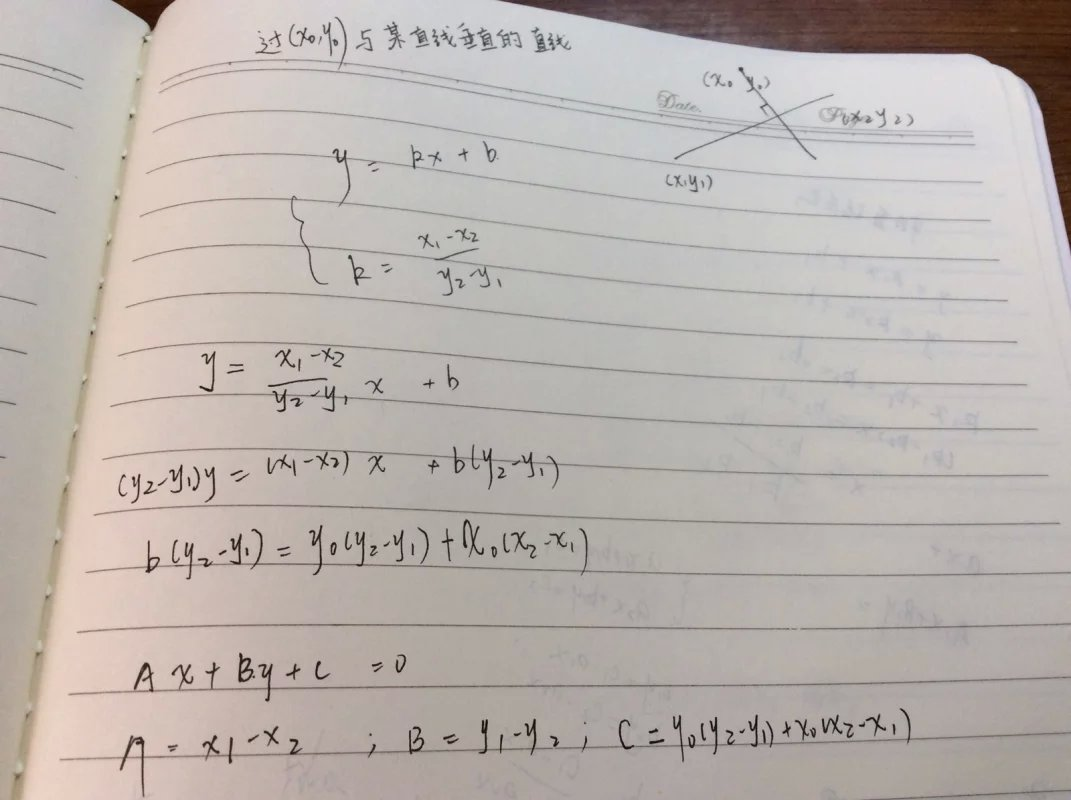编程实现：有了以上推导，编程实现上就显得容易很多。在编程实现的时候需要注意，我们求出交点，显然有多个返回值，所以在将各种操作(对称、求交点)写成函数的时候，可以用到指针或者引用。参考程序如下。(注：在推导过程中一般式按照ax+by+c=0推导，在编程的时候按照ax+by=c，这体现在代码上可能有正负号的微小差异，本质是相同的)#includevoid Intersect(double a1,double b1,double c1,double a2,double b2,double c2,double &x,double &y){x= (b2*c1 - b1*c2)/(a1*b2 - a2*b1);y= (a2*c1 - a1*c2)/(a2*b1 - a1*b2);         //计算两直线的交点}void line(double x1,double y1,double x2,double y2,double &a,double &b,double &c){a= y1 - y2;                                  //计算两点构成直线的参数a，b，cb = x2 -x1;c= x2*y1 - x1*y2;}intmain(){intT;doublex1,x2,y1,y2,x0,y0,x3,y3,a1,a2,b1,b2,c1,c2,x,y,a3,b3,c3;scanf("%d",&T);while(T--){scanf("%lf%lf%lf%lf%lf%lf%lf%lf",&x1,&y1,&x2,&y2,&x0,&y0,&x3,&y3);a1= x1 -x2;b1= y1 -y2;c1= x0*x1 - x0*x2 + y0*y1 - y0*y2;line(x1,y1,x2,y2,a2,b2,c2);Intersect(a1,b1,c1,a2,b2,c2,x,y);x+= x -x0;y+= y -y0;line(x,y,x3,y3,a3,b3,c3);Intersect(a3,b3,c3,a2,b2,c2,x,y);printf("%.3lf %.3lf\n",x,y);}}我们再来看一道关于求解多边形重心的问题。(Problem source:hdu1115)Problem DescriptionThere are many secret openings in the floor which are covered by a big heavy stone. When the stone is lifted up, a special mechanism detects this and activates poisoned arrows that are shot near the opening. The only possibility is to lift the stone very slowly and carefully. The ACM team must connect a rope to the stone and then lift it using a pulley. Moreover, the stone must be lifted all at once; no side can rise before another. So it is very important to find the centre of gravity and connect the rope exactly to that point. The stone has a polygonal shape and its height is the same throughout the whole polygonal area. Your task is to find the centre of gravity for the given polygon.InputThe input consists of T test cases. The number of them (T) is given on the first line of the input file. Each test case begins with a line containing a single integer N (3 <= N <= 1000000) indicating the number of points that form the polygon. This is followed by N lines, each containing two integers Xi and Yi (|Xi|, |Yi| <= 20000). These numbers are the coordinates of the i-th point. When we connect the points in the given order, we get a polygon. You may assume that the edges never touch each other (except the neighboring ones) and that they never cross. The area of the polygon is never zero, i.e. it cannot collapse into a single line.OutputPrint exactly one line for each test case. The line should contain exactly two numbers separated by one space. These numbers are the coordinates of the centre of gravity. Round the coordinates to the nearest number with exactly two digits after the decimal point (0.005 rounds up to 0.01). Note that the centre of gravity may be outside the polygon, if its shape is not convex. If there is such a case in the input data, print the centre anyway.题目大意：顺次给你n个顶点的坐标，让你找出n多边形的重心。数理分析：有了上面关于求解多边形面积的思维铺垫，这道题目将会轻松很多。我们知道，重心是来源于物理学中的概念。这里我们给出求几何体重心普适性的公式：①如果质量集中在顶点上，    n个顶点坐标为(xi,yi)，质量为mi，则重心X = ∑( xi×mi ) / ∑miY = ∑( yi×mi ) / ∑mi而特殊地，若每个点的质量相同，则X = ∑xi / nY = ∑yi / n②质量分布均匀(这里没有给出普适性的公式，因为在此算法中不需要)特殊地，质量均匀的三角形重心：X = ( x0 + x1 + x2 ) / 3Y = ( y0 + y1 + y2 ) / 3有了这些公式，我们下面要做的就是找到公式中字母在实际问题中代表的意义。先从整体的思路开始，秉承计算几何惯有的思维，这里还是要将n多边形分割成n - 2个三角形，基于三角形求重心的简便性，我们可以套用质量均匀分布情况下的三角形重心求解公式，此时，n多边形就转化成了质量集中在n - 2个三角形重心的图形了，此时我们再套用质量集中在顶点情况下的普适性公式。那么公式中的m代表什么呢？它是三角形的面积。我们立体化的看这些平面几何图形，假设他们有密度、厚度，肯定是一样的，那么这里质量就与平面中图形的面积成正比了。而对于三角形面积的计算，在上面也有所推导证明。编程实现：有了以上数理思维做铺垫，在编程实现的时候只需设置穷举正确的将n多边形分割成三角形即可。参考代码如下。#includeusing namespacestd;structpoint{doublex , y;};doublearea(point p1 , point p2 , point p3){return ((p2.x - p1.x) * (p3.y - p1.y) - (p3.x - p1.x)*(p2.y - p1.y))/2;}intmain(){point p1,p2,p3;intt , n;doubletemp , sumarea , xg , yg;scanf("%d",&t);while(t--){scanf("%d",&n);sumarea= xg = yg = 0;scanf("%lf%lf%lf%lf",&p1.x , &p1.y , &p2.x , &p2.y);for(int i = 2;i < n;i++){scanf("%lf%lf",&p3.x , &p3.y);temp=area(p1 , p2 , p3);xg+= (p1.x + p2.x + p3.x) *temp;yg+= (p1.y + p2.y + p3.y) *temp;sumarea+=temp;p2=p3;}xg= xg / sumarea / 3.0;yg= yg / sumarea / 3.0;printf("%.2lf %.2lf\n", xg , yg);}return 0;}再来看一道有关求多边形重心的问题(Problem source :pku 3855)DescriptionA new computer game has just arrived and as an active and always-in-the-scene player, you should finish it before the next university term starts. At each stage of this game, you have to shoot an enemy robot on its weakness point. The weakness point of a robot is always the “center of mass” of its 2D shape in the screen. Fortunately, all robot shapes are simple polygons with uniform density and you can write programs to calculate exactly the center of mass for each polygon.Let's have a more formal definition for center of mass (COM). The center of mass for a square, (also circle, and other symmetric shapes) is its center point. And, if a simple shape C is partitioned into two simple shapes A and B with areas SA and SB, then COM(C) (as a vector) can be calculated by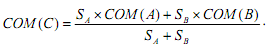As a more formal definition, for a simple shape A with area SA: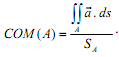InputThe input contains a number of robot definitions. Each robot definition starts with a line containing n, the number of vertices in robot’s polygon (n <= 100). The polygon vertices are specified in the next n lines (in either clockwise or counter-clock-wise order). Each of these lines contains two space-separated integers showing the coordinates of the corresponding vertex. The absolute value of the coordinates does not exceed 100. The case of n=0 shows the end of input and should not be processed.OutputThe ith line of the output should be of the form “Stage #i: x y” (omit the quotes), where (x,y) is the center of massfor the ith robot in the input. The coordinates must be rounded to exactly 6 digits after the decimal point.题目大意：依旧是给出顶点数n，然后依次给出n个顶点的坐标，让你求解n边形的重心。编程实现：虽然方法是和上面一题一模一样的，但是在答案输出的时却对精度有着不同的要求。这里如果我们的精度是小数点后6位，而某个答案是-0.0000001，此时如果不作处理会输出-0.000000，而正确答案应该是0.000000，虽然大小没变，但是oj返回一个错误结果。所以我们在这里要随着精度的变化对代码做出相应的改动。这里要求小数点后6位的精度，我们设置一个常量为0.000001，如果|x|<0.000001，我们就令x = 0.0，这样就会输出0.000000而不是-0.000000。参考代码如下。#include#includeusing namespacestd;const double eps = 1e-6;structpoint{doublex , y;};doublearea(point p1 , point p2 , point p3){return ((p2.x - p1.x) * (p3.y - p1.y) - (p3.x - p1.x)*(p2.y - p1.y))/2;}intmain(){point p1,p2,p3;intt , n;doubletemp , sumarea , xg , yg;int tt = 1;while(scanf("%d",&n)!=EOF &&n){sumarea= xg = yg = 0;scanf("%lf%lf%lf%lf",&p1.x , &p1.y , &p2.x , &p2.y);for(int i = 2;i < n;i++){scanf("%lf%lf",&p3.x , &p3.y);temp=area(p1 , p2 , p3);xg+= (p1.x + p2.x + p3.x) *temp;yg+= (p1.y + p2.y + p3.y) *temp;sumarea+=temp;p2=p3;}xg= xg / sumarea / 3.0;yg= yg / sumarea / 3.0;if(fabs(xg) xg= 0.0;if(fabs(yg) yg= 0.0;printf("Stage #%d: %.6f %.6f\n" , tt++, xg , yg);}return 0;}考虑这样一个问题，给定含有有限个元素的点集，我们如何变成计算能够覆盖所有点的最小的圆的圆心和半径，这也就是所谓的最小圆覆盖问题。(Problem source: zoj 1450)InputThe input contains several problems. The first line of each problem is a line   containing only one integer N which indicates the number of points to be covered.   The next N lines contain N points. Each point is represented by x and y coordinates   separated by a space. After the last problem, there will be a line contains   only a zero.OutputFor each input problem, you should give a one-line answer which contains three   numbers separated by spaces. The first two numbers indicate the x and y coordinates   of the result circle, and the third number is the radius of the circle. (use   escape sequence %.2f)编程实现：对于计算几何的问题，难点多在于编程实现自动化计算，在数理思维上不是很困难。实现最校园覆盖的算法有很多，这里介绍一种随机增量法。我们将设置三层遍历，依次遍历点集。第一层遍历，用于我们判断该点是否在目前构造的圆内，否则的话，此点作为新的圆心。第二层遍历，用于我们判断该点是否在目前构造的圆内，否则的话，此时选出的两点形成一条线段，中点即为圆心。第三层遍历，用于我们判断该点是否在目前构造的圆内，否则的话，此时遍历选出的三点的形成的三角形的外心是圆心。可能有人会疑惑这样编程的顺序，能否实现“最小”圆这一要求。进行第一层比较，两个点形成的圆必然比三个点(这三个点中包含前两个点)形成的圆要小，这是显而易见的。进行第二层比较，如果两个点形成了一个符合要求圆，那它是否是最小的呢？答案也是显然的，如果我们假设还存在另外一个更小的圆，那么此时开始的两个点将作为一条弦出现，如果这条弦是直径，那么这是个等大的圆；如果不是直径，那么这个圆的直径将大于这条弦，这与我们初始的假设相悖。所以我们得到结论，按照上述的过程，一旦构造出符合要求的圆，那么一定是最小的覆盖圆。在真正编程实现的时候还需要考虑精度问题，其具体的方法就是尽量减少* /运算的出现，因为其会产生误差，虽然看似极小，但是数据量一大之后，聚会产生较为明显差值从而导致错误。这里对于中垂线段的确定，我们给出简略的证明(针对遍历三个点是确定外接圆圆心的情况)。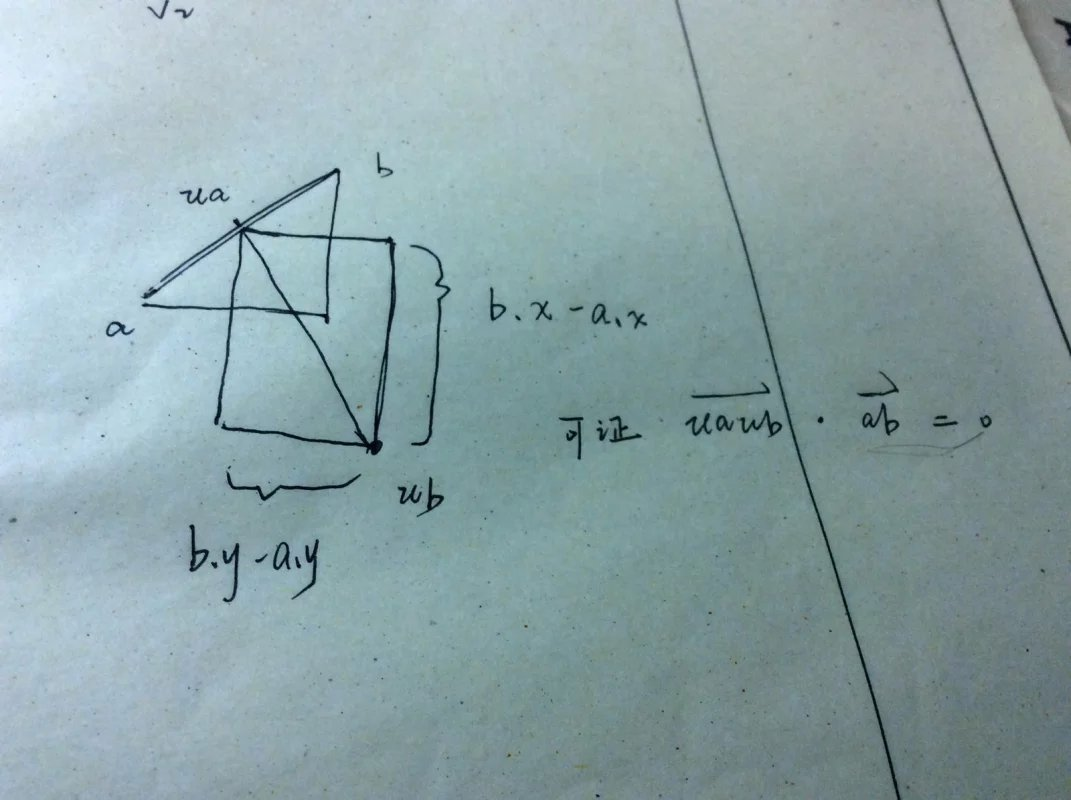如图所示，我们在线段ab及其重点ua的基础上构造出矩形，我们可通过全等证垂直，这样，中垂线段就用+ - 的形式表示出来，随后只需求两条线断的交点即可求出三点确定的圆的圆心。这样做相比于通过确立直线方程坐标然后联立得到方程组，很好的减少了出现精度差的运算次数。参考代码如下。#include#include#includeusing namespacestd;const int maxn = 505;const double eps = 1e-6;intn;doubler;structpoint{doublex , y;}p[maxn] , o;doubledis(point a , point b){return sqrt((a.x-b.x)*(a.x-b.x) + (a.y-b.y)*(a.y-b.y));}point Interesection(point u1,point u2,point v1,point v2){point ans=u1;double t = ((u1.x - v1.x) * (v1.y - v2.y) - (u1.y - v1.y) * (v1.x - v2.x)) /((u1.x- u2.x) * (v1.y - v2.y) - (u1.y - u2.y) * (v1.x -v2.x));ans.x+= (u2.x - u1.x) *t;ans.y+= (u2.y - u1.y) *t;return ans;    //高精度求线段交点，即为外接圆的圆心。}point Circum(point a , point b , point c){point ua,ub,va,vb;//求出两条中垂线段ua.x = ( a.x + b.x ) / 2;ua.y= ( a.y + b.y ) / 2;ub.x= ua.x - a.y +b.y;ub.y= ua.y + a.x -b.x;va.x= ( a.x + c.x ) / 2;va.y= ( a.y + c.y ) / 2;vb.x= va.x - a.y +c.y;vb.y= va.y + a.x -c.x;returnInteresection(ua,ub,va,vb);}point min_center(){inti , j , k;o= p;r= 0;for(i = 1;i < n;i++)  //三层循环，因为3点将确定一个圆{if(dis(o,p[i]) - r >eps ){o=p[i];r= 0;for(j = 0;j < i;j++){if(dis(p[j],o) - r >eps){o.x= (p[i].x + p[j].x)/2.0;o.y= (p[i].y + p[j].y)/2.0;r=dis(o,p[i]);for(k = 0;k < j;k++){if(dis(p[k],o) - r >eps){o=Circum(p[i],p[j],p[k]);r=dis(p[i],o);}}}}}}returno;}intmain(){while(scanf("%d",&n) , n){for(int i = 0;i < n;i++)scanf("%lf%lf",&p[i].x , &p[i].y);min_center();if(fabs(o.x) < 0.01)  o.x = 0.00;if(fabs(o.y) < 0.01)  o.y = 0.00;printf("%.2lf %.2lf %.2lf\n",o.x,o.y,r);}}那么基于对最小圆覆盖模型的认识，我们再来看一道与之有关的题目(Problem source : hdu4720)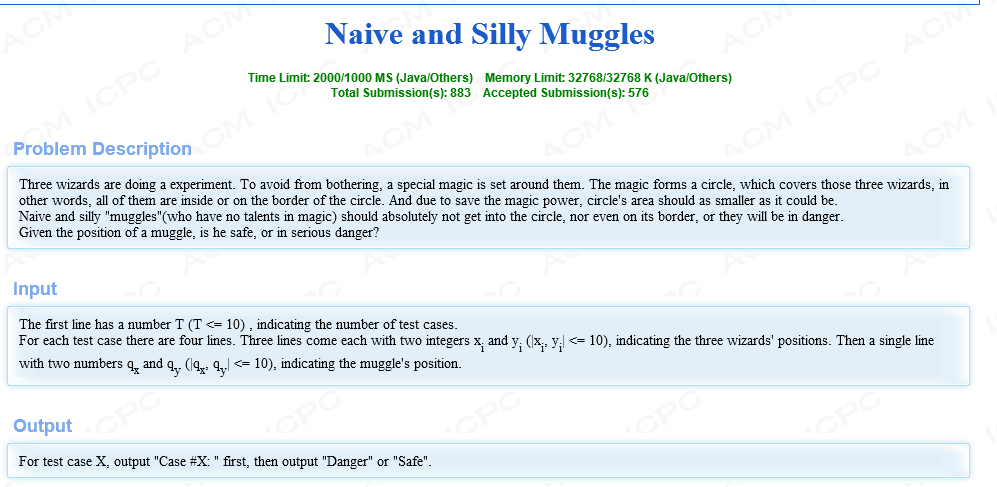题目大意：给你四个点，用前三个点来确定一个最小覆盖圆，然后判断第四个点是否在这个圆内。数理分析：这里其实是最小圆覆盖模型的超级简单版，把覆盖的n多边形变成了三角形。这里我们很容易看到，对于直角三角形和锐角三角形，我们要求的圆是外接圆，而对于钝角三角形，我们要求的则是一个覆盖圆。但是综合起来，我们都可以讲它们看成求三个点的最小覆盖圆。编程实现上没有什么难度，可以直接使用上面我们学习过的关于最小圆覆盖的代码(虽然有点大材小用)。参考代码如下。#include#include#includeusing namespacestd;const int maxn = 4;const double eps = 1e-6;intn;doubler;structpoint{doublex , y;}p[maxn] , o;doubledis(point a , point b){return sqrt((a.x-b.x)*(a.x-b.x) + (a.y-b.y)*(a.y-b.y));}point Interesection(point u1,point u2,point v1,point v2){point ans=u1;double t = ((u1.x - v1.x) * (v1.y - v2.y) - (u1.y - v1.y) * (v1.x - v2.x)) /((u1.x- u2.x) * (v1.y - v2.y) - (u1.y - u2.y) * (v1.x -v2.x));ans.x+= (u2.x - u1.x) *t;ans.y+= (u2.y - u1.y) *t;return ans;    //高精度求线段交点，即为外接圆的圆心。}point Circum(point a , point b , point c){point ua,ub,va,vb;//求出两条中垂线段ua.x = ( a.x + b.x ) / 2;ua.y= ( a.y + b.y ) / 2;ub.x= ua.x - a.y +b.y;ub.y= ua.y + a.x -b.x;va.x= ( a.x + c.x ) / 2;va.y= ( a.y + c.y ) / 2;vb.x= va.x - a.y +c.y;vb.y= va.y + a.x -c.x;returnInteresection(ua,ub,va,vb);}point min_center(){inti , j , k;o= p;r= 0;for(i = 1;i < n;i++)  //三层循环，因为3点将确定一个圆{if(dis(o,p[i]) - r >eps ){o=p[i];r= 0;for(j = 0;j < i;j++){if(dis(p[j],o) - r >eps){o.x= (p[i].x + p[j].x)/2.0;o.y= (p[i].y + p[j].y)/2.0;r=dis(o,p[i]);for(k = 0;k < j;k++){if(dis(p[k],o) - r >eps){o=Circum(p[i],p[j],p[k]);r=dis(p[i],o);}}}}}}returno;}intmain(){n= 3;intt;while(~scanf("%d",&t)){int tt = 1;while(t--){for(int i = 0;i < n;i++)scanf("%lf%lf",&p[i].x , &p[i].y);scanf("%lf%lf",&p.x,&p.y);min_center();printf("Case #%d:",tt++);if(dis(p,o) > r)  printf("Safe\n");else                 printf("Danger\n");}}}基于上面对最小圆覆盖问题的学习，我们再来看一道应用它的问题。(Problem source : hdu 3932)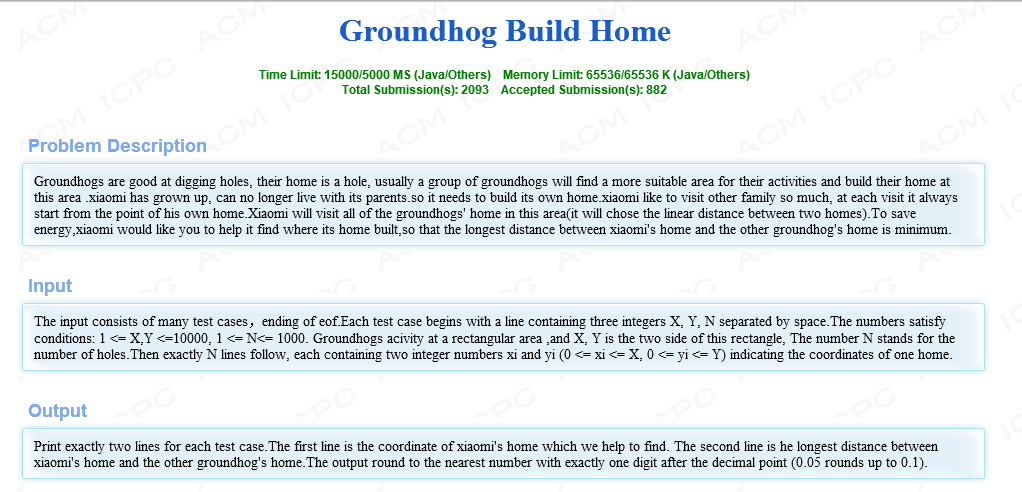题目大意：给出你n个点的坐标，你任取一个点，这个点到到这n个点中的某个点的距离都是存在最大值的，那么现在需要你找到这个最大值最小时的情况。输出这个点的坐标，并输出最小的最大距离。数理分析：我们很明显的能够分析到这里需要用到最小圆覆盖的模型。我们定性的来分析这种最值问题。如果我们n只有2个，那么显然要求这两个点构成线段的中点。如果n=3，我们则取这三个点构成三角形的外心。如果这n个点组成的n多边形的外接圆是存在的，那么这个外接圆的圆心就是我们要找的点。我们看到，为了使这个最大距离尽量小，就使n个点到这个点的距离的差值尽量小些。因为我们发现，如果我们移动该点，使得它靠近了i点，那么相应的会远离j点(也不尽然，这里是定性的分析)，为了使max(i,j)最小，我们就要一个“均衡受力”的点，所以，这个点应该是外接圆的圆心，因为它到各个点的距离都是一样的。那么如果不存在外接圆呢？那我们就要去相应的找最小覆盖圆了。有了这层数理分析，我们只要基于求解最小覆盖圆的模板，就可以很好的解决这个问题了。参考代码如下。(Ps:此题目对数据的要求是精确到小数点后一位，四舍五入，但是实际测评中并没有四舍五入，这里在代码中就没有体现四舍五入。)#include#include#includeusing namespacestd;const int maxn = 1005;const double eps = 1e-6;intn , x , y;doubler;structpoint{doublex , y;}p[maxn] , o;doubledis(point a , point b){return sqrt((a.x-b.x)*(a.x-b.x) + (a.y-b.y)*(a.y-b.y));}point Interesection(point u1,point u2,point v1,point v2){point ans=u1;double t = ((u1.x - v1.x) * (v1.y - v2.y) - (u1.y - v1.y) * (v1.x - v2.x)) /((u1.x- u2.x) * (v1.y - v2.y) - (u1.y - u2.y) * (v1.x -v2.x));ans.x+= (u2.x - u1.x) *t;ans.y+= (u2.y - u1.y) *t;return ans;    //高精度求线段交点，即为外接圆的圆心。}point Circum(point a , point b , point c){point ua,ub,va,vb;//求出两条中垂线段ua.x = ( a.x + b.x ) / 2;ua.y= ( a.y + b.y ) / 2;ub.x= ua.x - a.y +b.y;ub.y= ua.y + a.x -b.x;va.x= ( a.x + c.x ) / 2;va.y= ( a.y + c.y ) / 2;vb.x= va.x - a.y +c.y;vb.y= va.y + a.x -c.x;returnInteresection(ua,ub,va,vb);}point min_center(){inti , j , k;o= p;r= 0;for(i = 1;i < n;i++)  //三层循环，因为3点将确定一个圆{if(dis(o,p[i]) - r >eps ){o=p[i];r= 0;for(j = 0;j < i;j++){if(dis(p[j],o) - r >eps){o.x= (p[i].x + p[j].x)/2.0;o.y= (p[i].y + p[j].y)/2.0;r=dis(o,p[i]);for(k = 0;k < j;k++){if(dis(p[k],o) - r >eps){o=Circum(p[i],p[j],p[k]);r=dis(p[i],o);}}}}}}returno;}intmain(){while(~scanf("%d%d%d",&x,&y,&n)){for(int i = 0;i < n;i++)scanf("%lf%lf",&p[i].x , &p[i].y);min_center();printf("(%.1lf,%.1lf).\n",o.x,o.y);printf("%.1lf\n",r);}}在了解了最小矩阵覆盖、凸包、最小圆覆盖等覆盖类问题后，我们在这里再来看一道有关图形覆盖类问题。(Problem source 1077)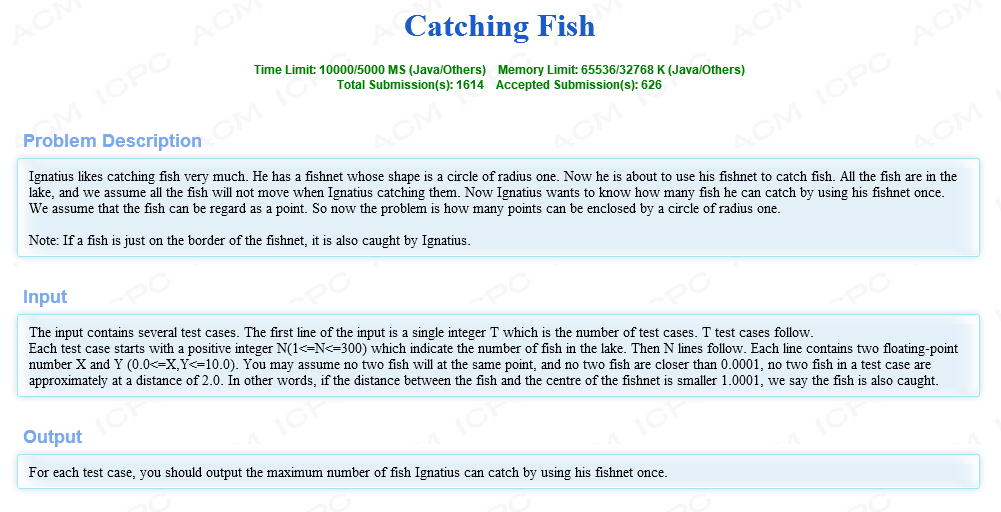题目大意：给出你n个平面内的点的坐标，然后让你用半径为1的圆去圈点，让你求出能够圈出的最大的点。数理分析：这道题在大的思路上肯定是要暴力穷举，这不难看出来，剩下我们要解决的就是针对每次穷举我们应该怎样处理。我们穷举要选取的是两个点，为什么是两个点呢？因为我们通过这两个点，再加上圆的半径，就可以确定单位圆的位置。什么？你说可能有两边的？那么你想对了，这的确是这道题目需要着力思考的一个地方。我们先不纠结于细节，单纯的拿出一个图来进行分析。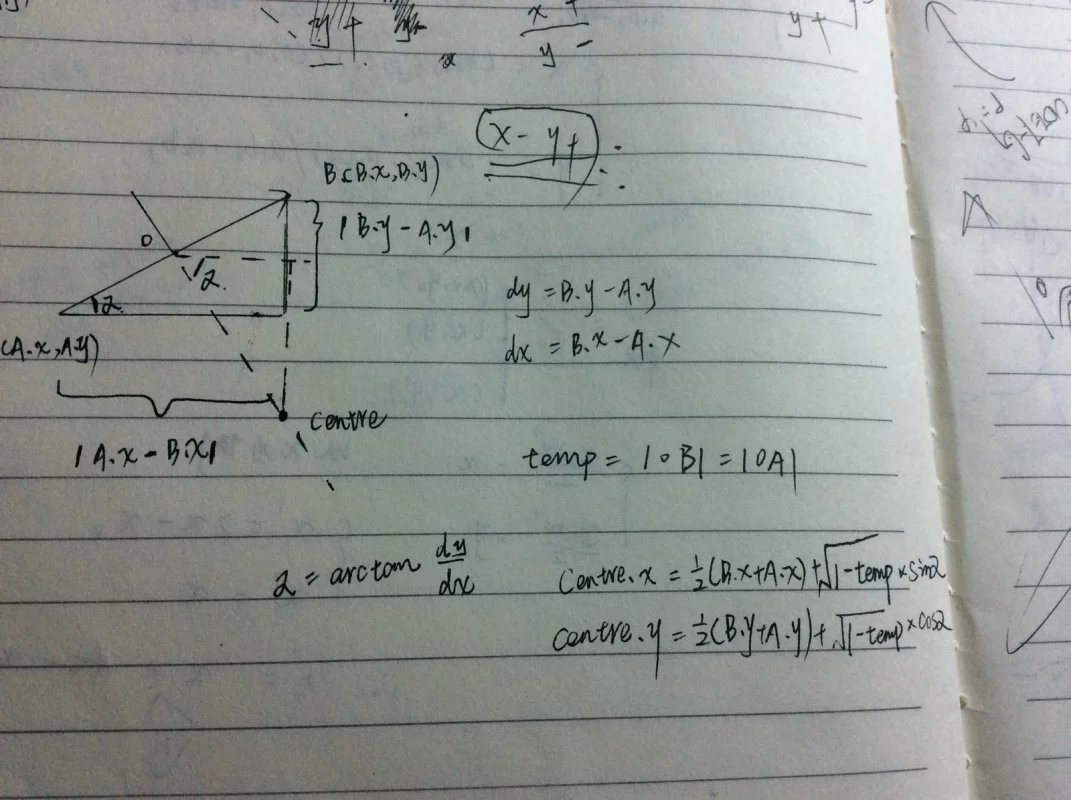通过画这一个图，我们可以简单的列出每次穷举的时候需要用到的计算公式，原理也十分简单，是最浅显的几何知识。那么现在的问题就是选取哪个半圆的问题。选取了半圆，我们用正余弦计算正负号的问题。这里做一个简单的思维铺垫，我们假定选取的两个点是有顺序的，即取的两个点A、B是有方向的,A->B，这样的话，我们在选圆的时候只需要选一个即可，因为在后面遍历的时候还会出现B->A，此时选出的圆将会对应着上次选择没有选到的那个圆，这要就做到了不重不漏。既然这样，我们开始基于一个点分析，加入我们选取的第一个点是A，那么就确立了关系A -> ? ，其中'?'就是我们下个要选取的字母。此时，其他字母相对于A有如下四种情况(之所以要分析这么详细是选取圆时，为了计算圆心，基于选取两点中点进行正余弦运算的正负号是不确定的)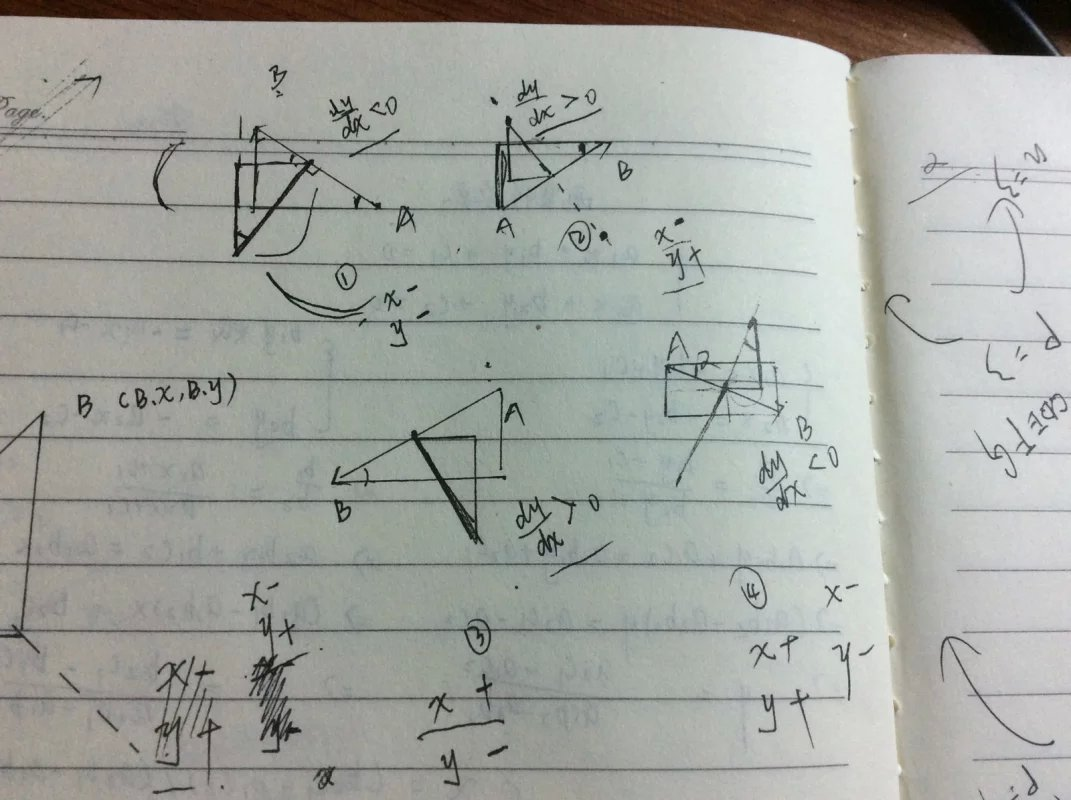其实细分到这，比较懒的一种做法就是四种情况分情况，对应四段代码，但是通常情况下呈现出的符号是有归纳性的规律的。这里答案其实呈现出多样性，我只就一种情况进行分析。在这四种情况中，dy / dx的正负我已标在图中。对于②③图，如果我们都选择直线AB左半平面的圆，此时取ang = arctan(-dy/dx)，那么这两个图呈现出-x，+y的运算规律，考虑到第四象限角的正余弦值，显然我们基于AB中点o，要进行-操作。同理，我们分析①④，选取右平面，就会得到同上的结论。这样我们就很好的将四种情况统一到了一起，并且不会漏不会重复。有了如上的数理思维，编程就很容易了。这里还有一个容易忽视的细节就是n = 1的临界情况分析，显然这种情况怎样都是可以圈出一个的，所以我们在进行暴力枚举的时候，应该注意比较大小的初始变量ans应该赋为1.参考代码如下。#include#includeusing namespacestd;const int maxn = 305;const double r = 1.0;const double eps = 1e-6;structpoint{doublex  ,y;}p[maxn];intn;double dis(int a , intb){return (p[a].x-p[b].x)*(p[a].x-p[b].x) + (p[a].y-p[b].y)*(p[a].y-p[b].y);}void get_centre(int a,intb){doubleox,oy,dx,dy,r,temp;ox=(p[a].x+p[b].x)/2;oy=(p[a].y+p[b].y)/2;dx=p[b].x-p[a].x;dy=p[b].y-p[a].y;p[n].x=ox;p[n].y=oy;temp=dis(n,b);r=sqrt(1.0-temp);if(fabs(dx) < eps)//竖直的情况p[n].x-=r;else{double ang=atan(-dy/dx);p[n].x-=r*sin(ang);     //这里给出的情况呼应上文的分析p[n].y-=r*cos(ang);}}intmain(){intT;inti , j  , k ;scanf("%d",&T);while(T--){scanf("%d",&n);for(i = 0;i < n;i++)scanf("%lf%lf",&p[i].x,&p[i].y);int Max , ans = 1;for(i = 0;i < n;i++){for(j = i + 1;j < n;j++){if(dis(i,j) >= 4)  continue;get_centre(i , j);for(k = 0,Max = 0;k < n;k++){if(n - k + Max <= ans)  break;double temp =sqrt(dis(k,n));if(temp <= 1.0001)Max++;}if(Max >ans)ans=Max;}}printf("%d\n",ans);}}基于上面对于单位圆点覆盖的问题的探讨，我们再来看一道类似的题目。(Problem source : pku 1379)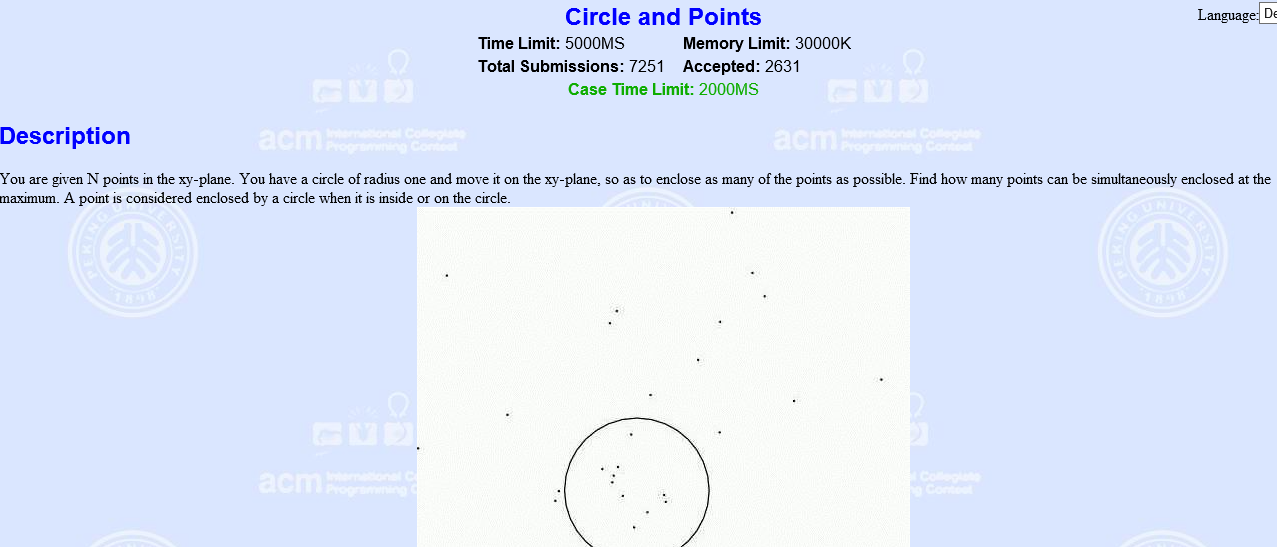题目阐述的很直接，就是给定一个含n个元素的点集，让你求找到用单位圆可以覆盖的最多的点是多少。基于对上题模型的思考，这里就可以简单的修改一下上面代码来实现了，这里就不再累述。参考系：《计算几何及应用》 金博 郭立
展开全文• 乐学数韵(ID/抖音：Vlxsy8 视频号/B站：乐学数韵)教研、解题、资源Q群：314559613,1078982440(2群)相关链接：平面解析几何的思维特征与研究方法高考中解析几何的那些“情怀”高中数学中简化解析几何运算的五个技巧骆...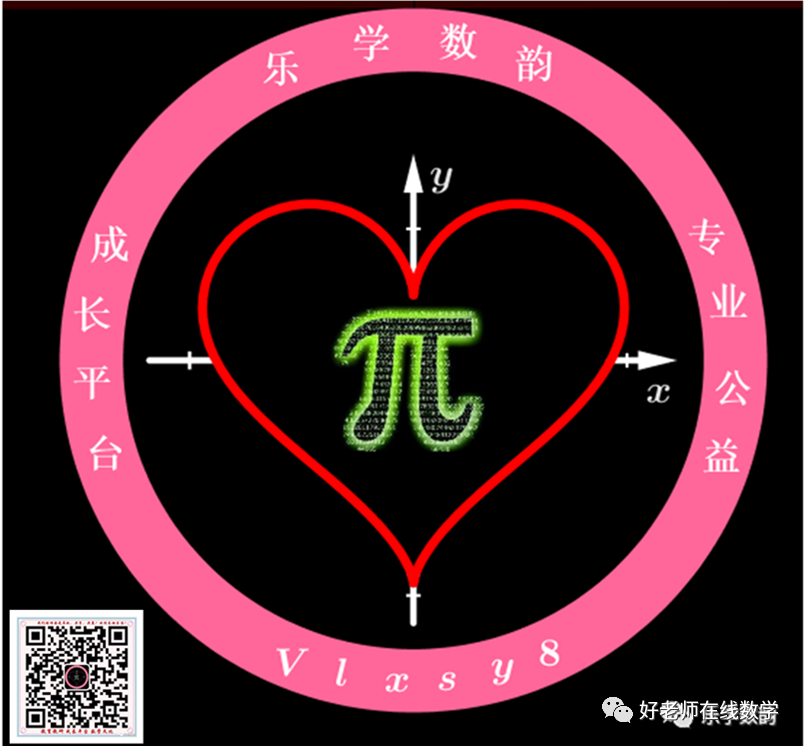乐学数韵(ID/抖音：Vlxsy8   视频号/B站：乐学数韵) 教研、解题、资源 Q群： 314559613  ,1078982440 (2群)相关链接：平面解析几何的思维特征与研究方法高考中解析几何的那些“情怀”高中数学中简化解析几何运算的五个技巧骆妃景 潘敬贞--聚焦核心素养，深度变式探究 ——一类解析几何面积最值问题的教学实录及反思高中数学：简化解析几何运算的5个技巧蔡玉书——2020年全国高考数学理科I卷解析几何试题赏析对2018年高考全国卷Ⅰ理科数学解析几何试题的拓展探究—兼谈核心素养下圆锥曲线的备考解析几何二轮复习策略——2020年高考压轴题突破之解析几何》降低解析几何问题运算量的必备8种策略平面几何在解三角形、向量、立体及解析的应用价值(更多解题方法归纳总结合集欢迎点击推文下方链接)众所周知,圆锥曲线试题是高考的一大“拦路虎”.不管是教师还是学生,在解决方法上往往过分强调“纯代数”的解法.即通过引进坐标系,建立点与坐标,曲线与方程之间的对应关系,将几何问题转化为代数问题,从而用代数方法研究几何问题.这些方法属于通性通法,固然是必须重点讲解和掌握的,但是它们的计算量偏大,很多考生就是因为冗长的计算半途而废.因此,如何另辟蹊径,减少运算量是高三一线老师必须认真思考的问题。圆锥曲线属于解析几何的内容,几何是学生在初中就已经接触到的知识.学生在初中就已经学习了平面几何的一些性质,再加上高中几何知识的补充与强化,学生有了较为全面的平面几何知识,较好的应用平面几何的能力.因此,在解决圆锥曲线的相关问题中,如果我们能够将平面几何的知识应用上去,抓住解析几何问题的本质特征“几何性”,结合圆锥曲线的知识进行求解,那么可以使问题的解决变得清爽简明,自然简约,收到事半功倍的效果.01类型一：三角形或梯形中位线的性质例1:2019年浙江卷理科第15题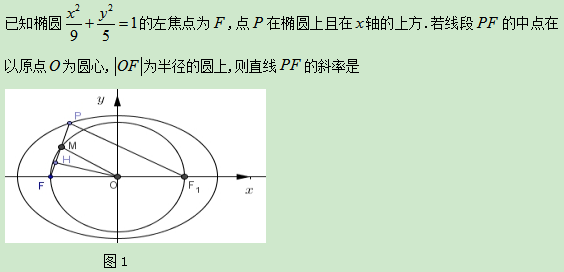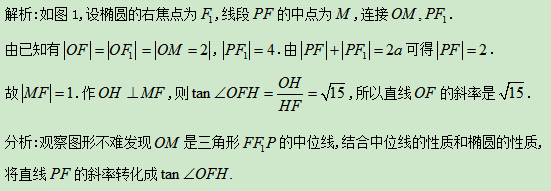例2.2017年全国II卷第16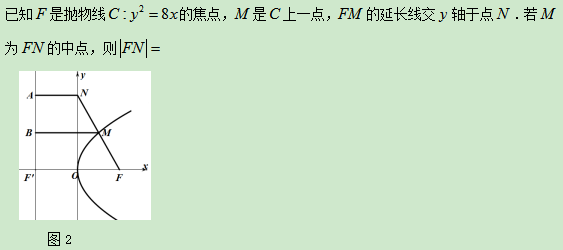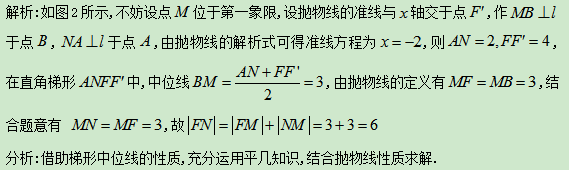02类型二：等腰三角形的性质或判定例3:2019年江苏卷理科第17题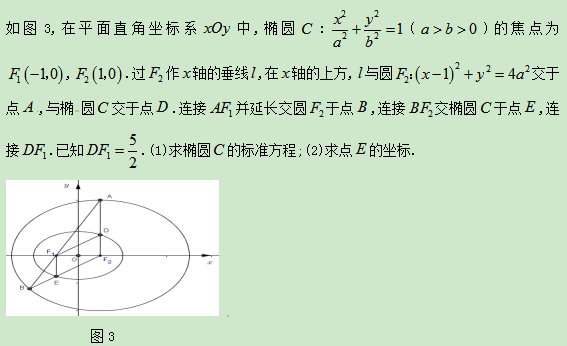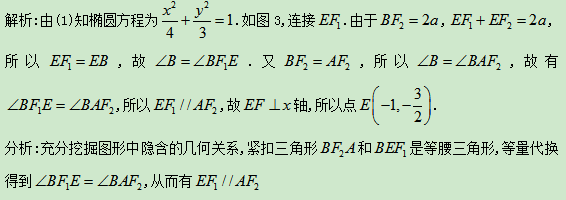例4:2016年全国I卷理科解几压轴试题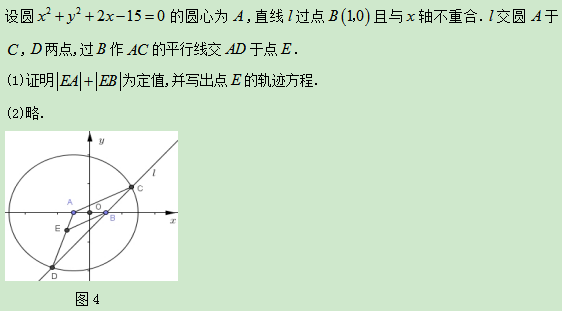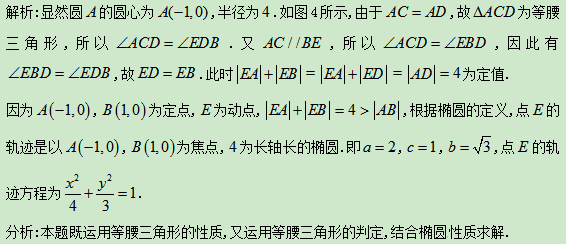03类型三：圆的性质例5:2019年全国I卷文科第21题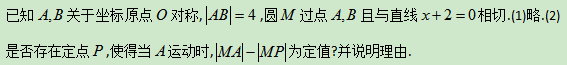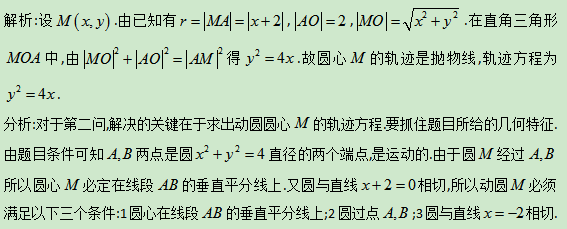例6.2018年江苏卷第12题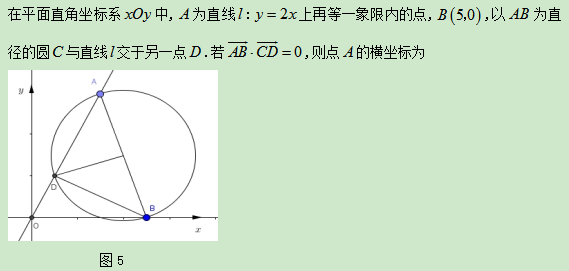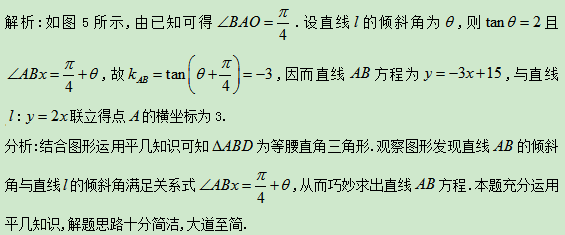04类型四：三角形内角平分线定理例7:2013年山东高考理科第22题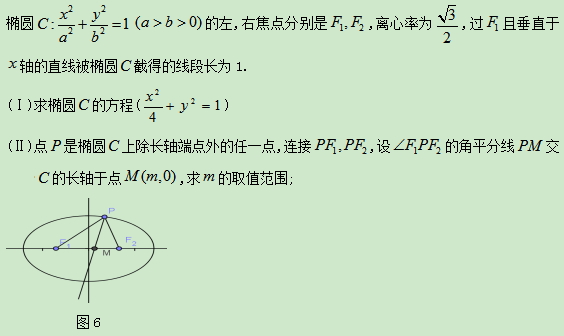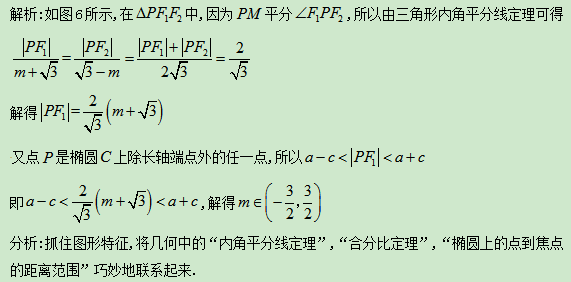05类型五：正弦定理或余弦定理例8:2012年辽宁高考理科第20题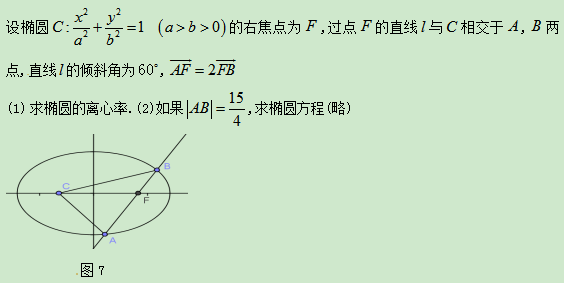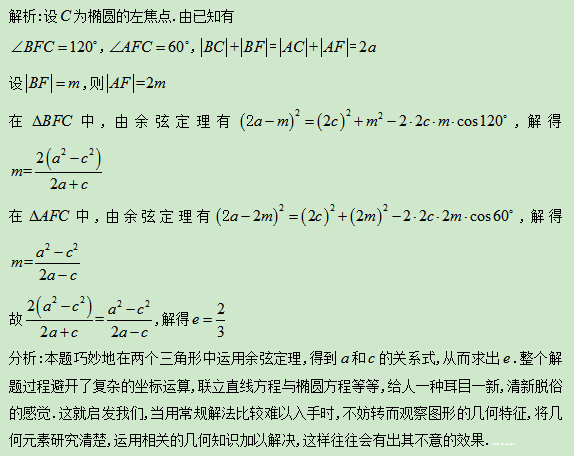06类型六：三角形三边长的关系例9:2012年四川高考理科第19题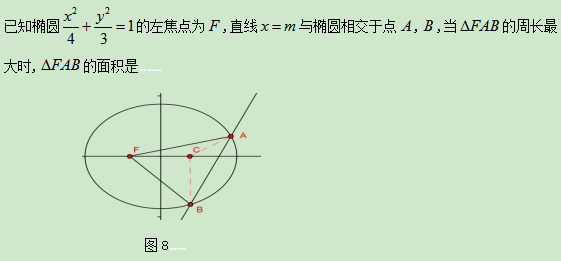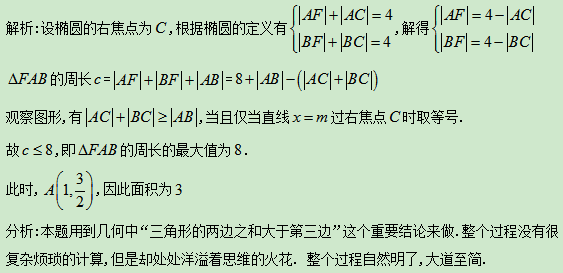07类型七：综合性问题例10.2017年全国I卷第15题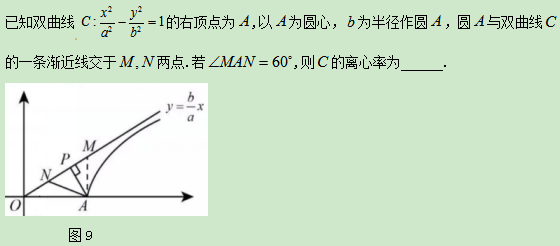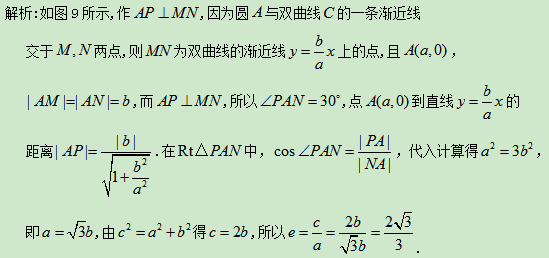例11:2013年全国卷高考理科第21题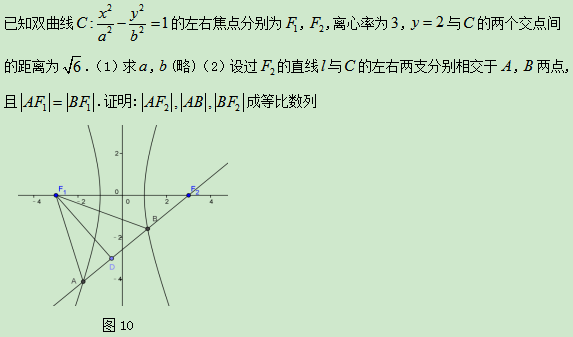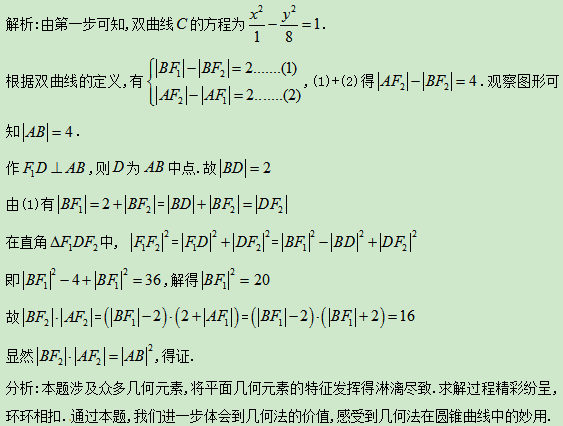例12:2019年全国I卷理科第16题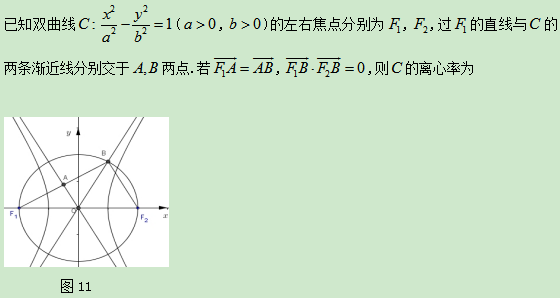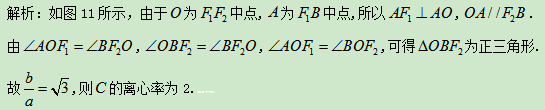以上资料来源于公众号：数学第六感假期弯道超车必备佳文:高中数学《立体几何》知识和方法合集高中数学《解析几何》系列线上视频合集高中数学《排列组合》微课视频合集高中数学《概率统计》微课视频合集高中数学《函数与导数》微课+线上课堂视频合集高考数学所有公式大汇编(文理合集)高中数学教学资料电子刊(教学视频+文本)大合集高中数学《数列》微课视频合集《高考数学全国卷解密》之三角形模型合集高中数学选修2-2《导数及其应用》微课视频合集完全免费||高考数学微课解题学习视频合集完全免费||高三数学视频课解题学习合集(二)2015-2018高考真题视频讲解合集！胜利在望||2020届高三最新模考试题合集(含下载方式)敬告：为防失联，请扫描关注《乐数》姊妹号《好老师在线数学》特别声明：非常时期，上述所有资源仅作公益分享，严禁用于商业用途，违者责任自负！版权归原作者和原出处所有.本文仅代表作者观点，本平台保持中立.如有侵权请联系删除，如需转载，请注明出处.来 源 ｜见上，转自高中数学王晖编 辑 ｜《好老师在线数学》  商务(合作)｜微mathcharm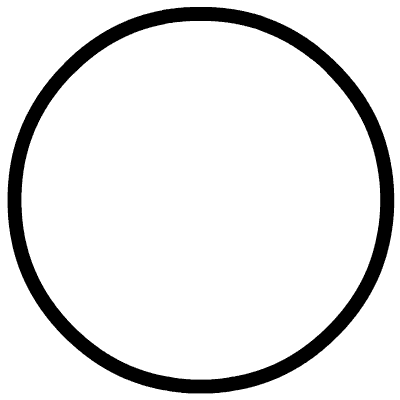戳原文，有惊喜！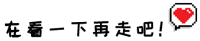展开全文• xml 解析几何 xml 解析几何 xml 解析几何
• 该PPT以一个清晰易懂的方式向未曾接触过空间解析几何的读者介绍其基本框架，质朴但却又不失实用性。这对于刚刚入门的读者或者刚刚接触多变量微积分学的读者来说应当有一定的指导作用。math
• 1. geoanalytic.py是解析几何的库 涉及几何的点 线 面 方程和 旋转变换等 2. geoelement.py是初等几何的库 涉及到几何的点 线 面 点到直线的距离 点到面的距离 三角形 四边形 3. matrix.py 矩阵及其计算 4. ...
• 空间解析几何与微分几何 第一章 向量 第二章 平面与直线 第三章 二次曲面 第四章 等距变换 正交变换 仿射变换 第五章 射影平面 第六章 练习题 第七章 局部曲线论 第八章 整体曲线论 第九章 局部曲面论
• In classical mathematics, analytic geometry, also known as coordinate geometry or Cartesian geometry, is the study of geometry using a ...解析几何，就是把几何放到指定的坐标系中去研究，从而构建起了...

In classical mathematics, analytic geometry, also known as coordinate geometry or Cartesian geometry, is the study of geometry using a coordinate system. – Wikipedia

解析几何，就是把几何放到指定的坐标系中去研究，从而构建起了代数与几何的桥梁。

根据使用的坐标系不同，基本可以分为

平面直角坐标系 Cartesian coordinates (in a plane or space) – 最常用的
极坐标系 Polar coordinates (in a plane)
柱面坐标系 Cylindrical coordinates (in a space)
球面坐标系 Spherical coordinates (in a space)

平面直角坐标系下的解析几何

感受一下无穷的公式 解析几何

展开全文• 解析几何学,是欧氏空间解析几何专著。由斜轴变换、斜轴画法、高维空间三部分组成。是PDF原版！
• 这是解析几何简明教程 文档本身十分清晰 适合初学解析几何的同学参考
• 解析几何中的类比1. (1)已知F1、F2是椭圆的两个焦点，其中a>b>0，Q是椭圆上一点（除长轴顶点），且∠F1QF2=θ，求：S△F1QF2=?(2)将上述结论类比到双曲线，并对命题加以证明.2.
• 这一讲我们学习解析几何，这是一种能解决几何难题的妙法。我们前面说了几何是最早出现的数学分支之一，一般来讲，人类的知识体系是从易到难建立的，但是几何学似乎要比后来出现的代数学来得难，这可能是人类逻辑推理...
• 尤承业编著的《解析几何》是学习几何学的入门教材。书中既讲解了空间解析几何的基本内容和方法（向量代数，仿射坐标系，空间的直线和平面，常见曲面等），等讲解了仿射几何学中的基本内容和思想（仿射坐标变换，二次...
• 莫斯科大学数学教程，几何讲义++第一学期++解析几何。该书是根据在莫斯科大学力学数学系开设几何学课程时的讲义写成的。内容包括线性空间、直线与平面的仿射几何、欧氏空间几何、二次曲线与二次曲面、仿射与射影几何...
• 为了弘扬浙江大学数学系的优良传统和学风，适应当代数学研究和教学的发展，2004年起浙江大学数学系组织力量对本科生课程设置和教材进行了重要改革，尤其是对数学系主干课程如数学分析、高等代数、解析几何、实变函数...
• 空间解析几何 苏步青 华宣积 忻元龙 张国梁 上海科学技术出版社 个人认为是最好的空间解析几何教材,很难找.
• 将线性代数与空间解析几何这两部分内容按其自身的内在联系合理地结合起来，使它们相互支持，前后呼应，成为一体。内容包括行列式、矩阵、几何向量、n维向量、空间中的平面与直线、线性方程组、特征值与特征向量、......